Contact Home

## 2D Liquid Simulator With Cellular Automaton in Unity

### Liquid Physics Using Cellular Automation

One interesting way to represent liquids in a grid based world, is to use a form of cellular automaton. You may have heard of cellular automata from the popular “Conway’s Game of Life”, where cells evolve based on a set of rules that they adhere to.

As the cells evolve, they create some interesting patterns, sometimes even chaotic in nature. For our liquid simulation, we are going to want to enforce some strict rules, so that we have much more control over the movement of our cells.

Take a look at the following gif. Notice how the liquids are all contained inside of grid cells. Each cell is capable of holding a value to indicate how much liquid is contained inside of it.

In this simulation, the cells attempt to disperse their liquid into their neighboring cells. It will do this by following rules that we will set for them. In each iteration of our simulation, every cell that contains liquid will execute the same set of rules.

### Rule #1: Flowing Into Bottom Neighboring Cell

First, a cell will check to see if it is capable of flowing downwards into its bottom neighbor. This makes sense as we want liquid to fall down as if it was affected by gravity.

The amount of liquid that is allowed to flow into another cell is calculated based on the amount of liquid already in the source and destination cells. Liquid will only flow if the destination cell has less liquid than the source cell.

Once we have calculated the amount of liquid to flow, we make the adjustment to the liquid value of both cells to reflect the change. The images below (from left to right) depict how the liquid behaves, showing one iteration of the simulation per image.

The liquid falls into the cell below in each iteration, until it is unable to fall any more:

### Rule #2: Flowing Into Left and Right Neighboring Cells

Once the cell has finished executing the first rule, it will then attempt to distribute its remaining liquid towards the left or right neighboring cells. Again, the amount of liquid that is allowed to flow is dependent on the current amount of liquid in each cell.

In the following images, you see that the liquid moves down during the first iteration. During the second iteration, it can no longer flow downwards, therefore it flows into the adjacent cell that is available to its right:

If both neighboring left and right cells are available to accept liquid, then the cell will flow in both directions in the same iteration:

### Rule #3: Flowing Upwards with Pressure

After the cell has executed both Rule #1 and Rule #2, it will then verify if it still contains more liquid than the maximum amount allowed. If it does contain more liquid, then the cell becomes pressurized. A pressurized cell will be allowed to flow upwards if possible. This behavior is illustrated below:

### Determining the Flow Direction of each Cell

As we iterate over each of our cells that contain liquid, and apply the above rules, we can also keep track of the last iterations flow direction for each of our cells. Doing so, will allow us to know in what direction each cell is flowing at all times.

### Rendering Falling Liquids

Liquids that are flowing downwards do not render very nicely, as they sometimes only contain a small amount of liquid in the cells. Rendering the amount of liquid directly in these cells can look somewhat strange:

If we add a check to see if the liquid flowing downwards, we can render these cells as having a full unit of liquid. This is mainly to make the simulation render a little nicer. Doing so, would render the liquids like this:

### Conclusion

Although this type of liquid simulation is a great for grid based solutions, in order for the liquid to be fluid, it requires a high iteration rate on each frame. By increasing the iteration count, you can adjust the speed at which the liquid moves making it much more realistic, at the expense of more CPU cycles per frame.

```// Run our liquid simulation
for (int n = 0; n < Iterations; n++)
LiquidSimulator.Simulate (ref Cells);
```

Finding a balance that works for you will be an important step to take when implementing a system like this.

### Try the Demo

Check out the WebGL demo here.

Left Click to place/remove walls. Right click to place liquid.

Liquid Simulator Demo

### Source Code

Source code has been published on Github:

https://github.com/jongallant/LiquidSimulator

References:

## Procedurally Generating Wrapping World Maps in Unity C# – Part 4

Check out Part 1, Part 2 and Part 3 of this series if you haven’t already. This is a continuation of those articles.

In the previous articles:

### Generating Biomes

Biomes are a way of classifying terrain types. Our biome generator will be based on the ever popular Whittaker’s model, where biomes are classified based on precipitation and temperature. Since we already generated a heat map and a moisture map for our world, determining our biomes will be pretty easy. Whittaker’s classification scheme is represented in the following diagram: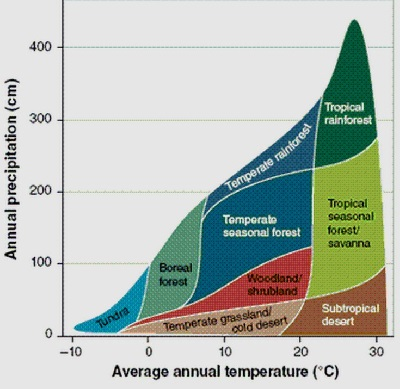We can identify different biome types based on a given temperature and moisture level. First, we can easily create a new enumeration that will store these biome types:

```public enum BiomeType
{
Desert,
Savanna,
TropicalRainforest,
Grassland,
Woodland,
SeasonalForest,
TemperateRainforest,
BorealForest,
Tundra,
Ice
}
```

Then, we need to create a table that will tell us what biome type to use based on the temperature and humidity. We already have a HeatType, and a MoistureType. Each of these enumerations have 6 defined types. A table was created to match each of these types with Whittaker’s diagram, represented below: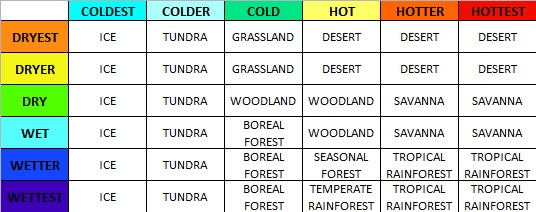In order to easily look up this data in code, we can easily just recreate this table as a two-dimensional array. This looks like the following:

```BiomeType[,] BiomeTable = new BiomeType[6,6] {
//COLDEST        //COLDER          //COLD                  //HOT                          //HOTTER                       //HOTTEST
{ BiomeType.Ice, BiomeType.Tundra, BiomeType.Grassland,    BiomeType.Desert,              BiomeType.Desert,              BiomeType.Desert },              //DRYEST
{ BiomeType.Ice, BiomeType.Tundra, BiomeType.Grassland,    BiomeType.Desert,              BiomeType.Desert,              BiomeType.Desert },              //DRYER
{ BiomeType.Ice, BiomeType.Tundra, BiomeType.Woodland,     BiomeType.Woodland,            BiomeType.Savanna,             BiomeType.Savanna },             //DRY
{ BiomeType.Ice, BiomeType.Tundra, BiomeType.BorealForest, BiomeType.Woodland,            BiomeType.Savanna,             BiomeType.Savanna },             //WET
{ BiomeType.Ice, BiomeType.Tundra, BiomeType.BorealForest, BiomeType.SeasonalForest,      BiomeType.TropicalRainforest,  BiomeType.TropicalRainforest },  //WETTER
{ BiomeType.Ice, BiomeType.Tundra, BiomeType.BorealForest, BiomeType.TemperateRainforest, BiomeType.TropicalRainforest,  BiomeType.TropicalRainforest }   //WETTEST
};
```

To make the lookup even easier, we will add a new function that will return the biome type of any tile. This part is quite simple, as each tile already has an associated heat and moisture type.

```public BiomeType GetBiomeType(Tile tile)
{
return BiomeTable [(int)tile.MoistureType, (int)tile.HeatType];
}
```

This check is done for every single tile, and assigns all of our map’s biome zones.

```private void GenerateBiomeMap()
{
for (var x = 0; x < Width; x++) {
for (var y = 0; y < Height; y++) {

if (!Tiles[x, y].Collidable) continue;

Tile t = Tiles[x,y];
t.BiomeType = GetBiomeType(t);
}
}
}
```

Great, so now all of the biomes are assigned. However, we have no way of seeing them yet. Our next step, is to assign a color for each Biome type. This will allow us to visually associate each biome region, so that we can represent them in an image. The colors I chose are as follows: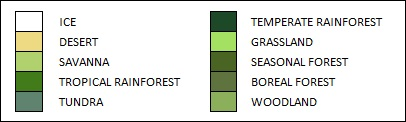These color values are plugged into the TextureGenerator class, along with the Biome texture generation code:

```	//biome map
private static Color Ice = Color.white;
private static Color Desert = new Color(238/255f, 218/255f, 130/255f, 1);
private static Color Savanna = new Color(177/255f, 209/255f, 110/255f, 1);
private static Color TropicalRainforest = new Color(66/255f, 123/255f, 25/255f, 1);
private static Color Tundra = new Color(96/255f, 131/255f, 112/255f, 1);
private static Color TemperateRainforest = new Color(29/255f, 73/255f, 40/255f, 1);
private static Color Grassland = new Color(164/255f, 225/255f, 99/255f, 1);
private static Color SeasonalForest = new Color(73/255f, 100/255f, 35/255f, 1);
private static Color BorealForest = new Color(95/255f, 115/255f, 62/255f, 1);
private static Color Woodland = new Color(139/255f, 175/255f, 90/255f, 1);

public static Texture2D GetBiomeMapTexture(int width, int height, Tile[,] tiles, float coldest, float colder, float cold)
{
var texture = new Texture2D(width, height);
var pixels = new Color[width * height];

for (var x = 0; x < width; x++)
{
for (var y = 0; y < height; y++)
{
BiomeType value = tiles[x, y].BiomeType;

switch(value){
case BiomeType.Ice:
pixels[x + y * width] = Ice;
break;
case BiomeType.BorealForest:
pixels[x + y * width] = BorealForest;
break;
case BiomeType.Desert:
pixels[x + y * width] = Desert;
break;
case BiomeType.Grassland:
pixels[x + y * width] = Grassland;
break;
case BiomeType.SeasonalForest:
pixels[x + y * width] = SeasonalForest;
break;
case BiomeType.Tundra:
pixels[x + y * width] = Tundra;
break;
case BiomeType.Savanna:
pixels[x + y * width] = Savanna;
break;
case BiomeType.TemperateRainforest:
pixels[x + y * width] = TemperateRainforest;
break;
case BiomeType.TropicalRainforest:
pixels[x + y * width] = TropicalRainforest;
break;
case BiomeType.Woodland:
pixels[x + y * width] = Woodland;
break;
}

// Water tiles
if (tiles[x,y].HeightType == HeightType.DeepWater) {
pixels[x + y * width] = DeepColor;
}
else if (tiles[x,y].HeightType == HeightType.ShallowWater) {
pixels[x + y * width] = ShallowColor;
}

// draw rivers
if (tiles[x,y].HeightType == HeightType.River)
{
float heatValue = tiles[x,y].HeatValue;

if (tiles[x,y].HeatType == HeatType.Coldest)
pixels[x + y * width] = Color.Lerp (IceWater, ColdWater, (heatValue) / (coldest));
else if (tiles[x,y].HeatType == HeatType.Colder)
pixels[x + y * width] = Color.Lerp (ColdWater, RiverWater, (heatValue - coldest) / (colder - coldest));
else if (tiles[x,y].HeatType == HeatType.Cold)
pixels[x + y * width] = Color.Lerp (RiverWater, ShallowColor, (heatValue - colder) / (cold - colder));
else
pixels[x + y * width] = ShallowColor;
}

if (tiles[x,y].HeightType >= HeightType.Shore && tiles[x,y].HeightType != HeightType.River)
{
pixels[x + y * width] = Color.Lerp (pixels[x + y * width], Color.black, 0.35f);
}
}
}

texture.SetPixels(pixels);
texture.wrapMode = TextureWrapMode.Clamp;
texture.Apply();
return texture;
}

```

Rendering these biome maps, gives us these beautiful wrapping world maps.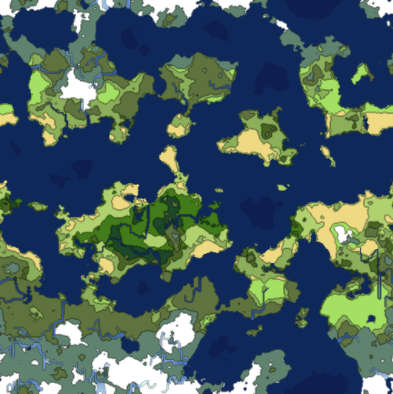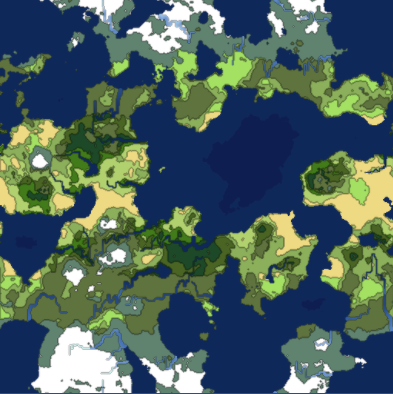### Generating Spherical Maps

Up until this point, we have created worlds that wrap around the X and Y axis. These maps are great for games, as the data can easily be rendered as a game map.

If you wanted to project these wrappable textures onto a sphere, it would not look right. In order to make our world fit a sphere, we need to write a spherical texture generator. In this section, we will add this functionality to the worlds we have been generating.

The spherical generation is going to differ slightly from the wrappable generator, as it will require different noise patterns, and texture mapping. For this reason, we are going to branch off the generator class into two new sub classes, WrappableWorldGenerator and SphericalWorldGenerator, both will inherit from their base Generator class.

This will allow us to have shared core functionality, while providing custom extended features for each generator type.

The original Generator class will become abstract, as well as some of its functions:

```    protected abstract void Initialize();
protected abstract void GetData();

protected abstract Tile GetTop(Tile tile);
protected abstract Tile GetBottom(Tile tile);
protected abstract Tile GetLeft(Tile tile);
protected abstract Tile GetRight(Tile tile);
```

The Initialize() and GetData() functions that we currently have, are tailored for the Wrappable worlds, therefore, we are going to have to implement new ones for the Spherical generator. We are also going to have to create new Tile fetch classes, as we are only going to be wrapping on the x-axis with these spherical projections.

We initialize the noise similarly, however, with one main difference. The heat map in this particular generator is not going to be wrapping on the y-axis. Because of this, we cannot create a proper gradient that we can multiply. Instead, we will manually do this, while generating the data.

```protected override void Initialize()
{
HeightMap = new ImplicitFractal (FractalType.MULTI,
BasisType.SIMPLEX,
InterpolationType.QUINTIC,
TerrainOctaves,
TerrainFrequency,
Seed);

HeatMap = new ImplicitFractal(FractalType.MULTI,
BasisType.SIMPLEX,
InterpolationType.QUINTIC,
HeatOctaves,
HeatFrequency,
Seed);

MoistureMap = new ImplicitFractal (FractalType.MULTI,
BasisType.SIMPLEX,
InterpolationType.QUINTIC,
MoistureOctaves,
MoistureFrequency,
Seed);
}
```

The GetData function is going to change dramatically. We are now going to go back to sampling 3D noise. The noise will be sampled with the help of a latitude and longitude coordinate system.

I looked at how libnoise did their spherical mapping, and applied the same concept here. The main code is the following, which converts the latitude and longitude coordinates, into 3D spherical cartesian map coordinates.

```void LatLonToXYZ(float lat, float lon, ref float x, ref float y, ref float z)
{
float r = Mathf.Cos (Mathf.Deg2Rad * lon);
x = r * Mathf.Cos (Mathf.Deg2Rad * lat);
y = Mathf.Sin (Mathf.Deg2Rad * lon);
z = r * Mathf.Sin (Mathf.Deg2Rad * lat);
}
```

The GetData function will then loop through all coordinates, using this conversion method to generate the map data. We sample the heat, height and moisture data using this method. The biome map is generated the same way as before, from the resulting moisture and heat maps.

```protected override void GetData()
{
HeightData = new MapData (Width, Height);
HeatData = new MapData (Width, Height);
MoistureData = new MapData (Width, Height);

// Define our map area in latitude/longitude
float southLatBound = -180;
float northLatBound = 180;
float westLonBound = -90;
float eastLonBound = 90;

float lonExtent = eastLonBound - westLonBound;
float latExtent = northLatBound - southLatBound;

float xDelta = lonExtent / (float)Width;
float yDelta = latExtent / (float)Height;

float curLon = westLonBound;
float curLat = southLatBound;

// Loop through each tile using its lat/long coordinates
for (var x = 0; x < Width; x++) {

curLon = westLonBound;

for (var y = 0; y < Height; y++) {

float x1 = 0, y1 = 0, z1 = 0;

// Convert this lat/lon to x/y/z
LatLonToXYZ (curLat, curLon, ref x1, ref y1, ref z1);

// Heat data
float sphereValue = (float)HeatMap.Get (x1, y1, z1);
if (sphereValue > HeatData.Max)
HeatData.Max = sphereValue;
if (sphereValue < HeatData.Min)
HeatData.Min = sphereValue;
HeatData.Data [x, y] = sphereValue;

// Adjust heat based on latitude
float coldness = Mathf.Abs (curLon) / 90f;
float heat = 1 - Mathf.Abs (curLon) / 90f;
HeatData.Data [x, y] += heat;
HeatData.Data [x, y] -= coldness;

// Height Data
float heightValue = (float)HeightMap.Get (x1, y1, z1);
if (heightValue > HeightData.Max)
HeightData.Max = heightValue;
if (heightValue < HeightData.Min)
HeightData.Min = heightValue;
HeightData.Data [x, y] = heightValue;

// Moisture Data
float moistureValue = (float)MoistureMap.Get (x1, y1, z1);
if (moistureValue > MoistureData.Max)
MoistureData.Max = moistureValue;
if (moistureValue < MoistureData.Min)
MoistureData.Min = moistureValue;
MoistureData.Data [x, y] = moistureValue;

curLon += xDelta;
}
curLat += yDelta;
}
}
```

Giving us our height map, heat map, moisture map, and biome map (respectively):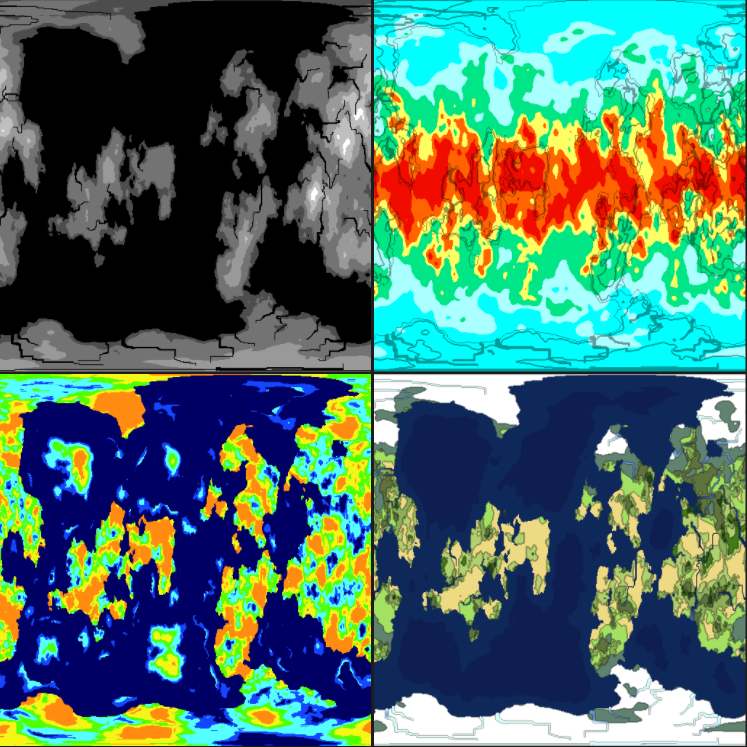Notice that the maps curve near the corners. This is intentional, as it is how the spherical projection works. Let’s apply the biome texture onto a sphere and see how it looks: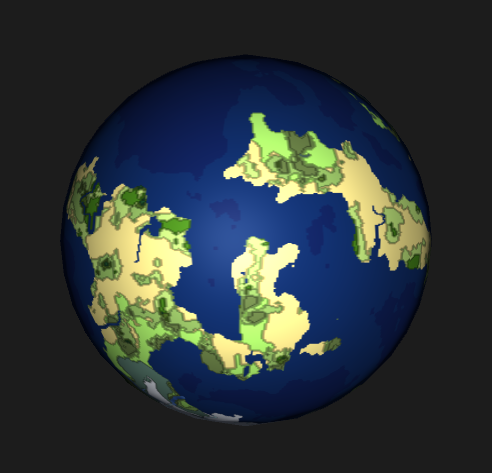Not a bad start. Now, you have have noticed that our height map is now black and white. This was done on purpose, as we are going to be using it as the height map for our sphere’s shader. We are also going to need a bump map to provide some extra effect. In order to generate the bump map, we will first render a black and white texture that represents what we want our distortion to be. This texture will then be processed into the actual bump map with the following code:

```    public static Texture2D CalculateBumpMap(Texture2D source, float strength)
{
Texture2D result;
float xLeft, xRight;
float yUp, yDown;
float yDelta, xDelta;
var pixels = new Color[source.width * source.height];
strength = Mathf.Clamp(strength, 0.0F, 10.0F);
result = new Texture2D(source.width, source.height, TextureFormat.ARGB32, true);

for (int by = 0; by < result.height; by++)
{
for (int bx = 0; bx < result.width; bx++)
{
xLeft = source.GetPixel(bx - 1, by).grayscale * strength;
xRight = source.GetPixel(bx + 1, by).grayscale * strength;
yUp = source.GetPixel(bx, by - 1).grayscale * strength;
yDown = source.GetPixel(bx, by + 1).grayscale * strength;
xDelta = ((xLeft - xRight) + 1) * 0.5f;
yDelta = ((yUp - yDown) + 1) * 0.5f;

pixels[bx + by * source.width] = new Color(xDelta, yDelta, 1.0f, yDelta);
}
}

result.SetPixels(pixels);
result.wrapMode = TextureWrapMode.Clamp;
result.Apply();
return result;
}
```

Feeding this function the texture on the left, gives us our bump map, represented on the right: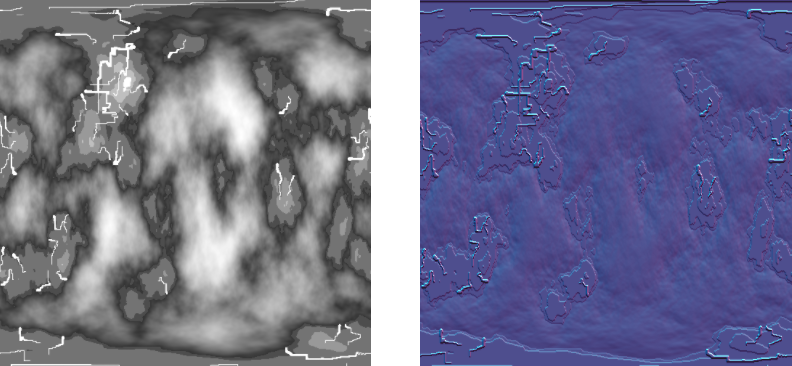Now, if we apply this bump map along with the height map, onto our sphere via the standard shader, we get the following: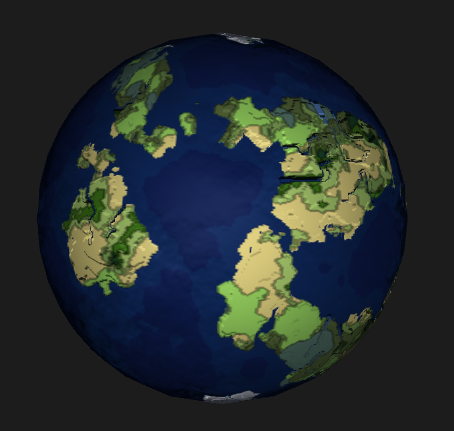For some extra effect, we are now going to add some cloud layers. We can generate clouds with noise very easily, so why not. We will use a billow noise module to represent our clouds.

We are going to add two cloud layers to give it some depth. The code for the cloud noise generator is:

```        Cloud1Map = new ImplicitFractal(FractalType.BILLOW,
BasisType.SIMPLEX,
InterpolationType.QUINTIC,
5,
1.65f,
Seed);

Cloud2Map = new ImplicitFractal (FractalType.BILLOW,
BasisType.SIMPLEX,
InterpolationType.QUINTIC,
6,
1.75f,
Seed);
```

We grab the data the same way. The cloud texture generator is just a simple color lerp from white to transparent white. We cut off the clouds at a set value, making everything else transparent. The code for the cloud texture generation is:

```public static Texture2D GetCloudTexture(int width, int height, Tile[,] tiles, float cutoff)
{
var texture = new Texture2D(width, height);
var pixels = new Color[width * height];

for (var x = 0; x < width; x++)
{
for (var y = 0; y < height; y++)
{
if (tiles[x,y].CloudValue > cutoff)
pixels[x + y * width] = Color.Lerp(new Color(1f, 1f, 1f, 0), Color.white, tiles[x,y].CloudValue);
else
pixels[x + y * width] = new Color(0,0,0,0);
}
}

texture.SetPixels(pixels);
texture.wrapMode = TextureWrapMode.Clamp;
texture.Apply();
return texture;
}
```

With this, we can generate two different cloud textures. Again, these textures were sampled to be spherical, which will warp near the corners: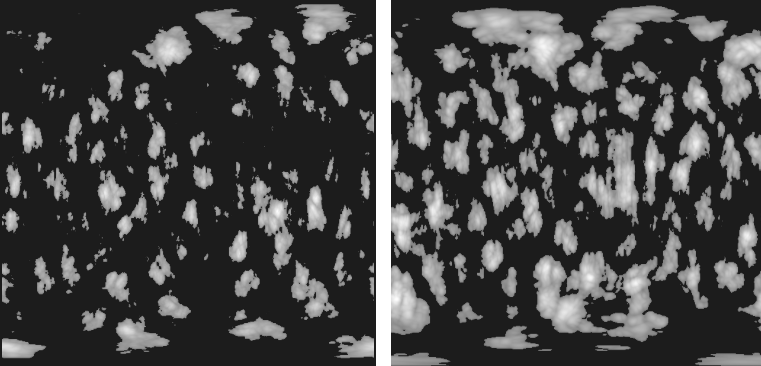Next, two new sphere meshes were added, that are slightly larger than the original sphere. Applying the cloud textures, to the standard shader with a fade effect, gives us some decent looking cloud coverage: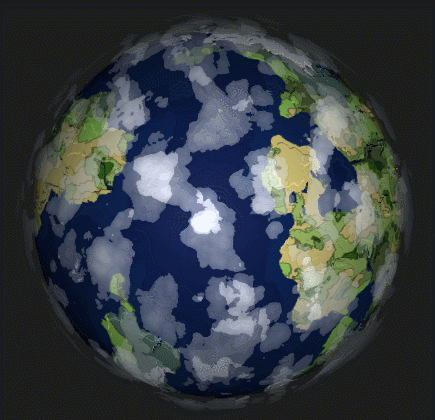Finally, here is a screenshot of all the textures that were generated, and used to create the final rendering of the planet: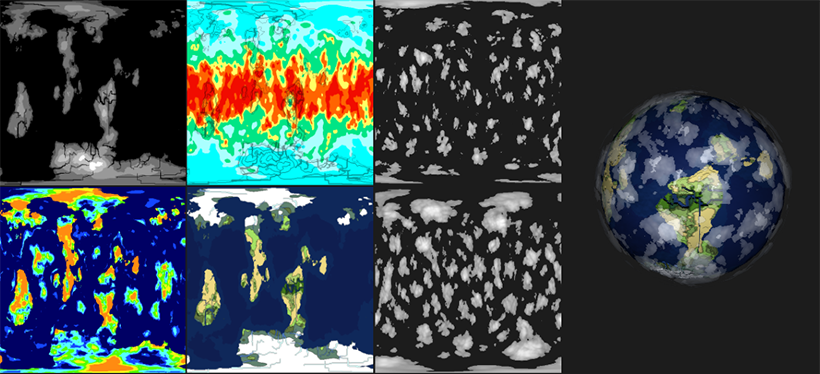That wraps it up for this tutorial series. You can get the full source code for this project on github.

## Procedurally Generating Wrapping World Maps in Unity C# – Part 3

Check out Part 1 and Part 2 of this series if you haven’t already. This is a continuation of that article.

In the previous articles:

In Part 4:

### Generating the Heat Map

A heat map defines the temperature of our generated world. The heat map we are going to create will be based on latitude and height. The latitude portion, can be done with a simple noise gradient. The Accidental Noise library provides us with this function:

```ImplicitGradient gradient = new ImplicitGradient (1, 1, 1, 0, 1, 1, 1, 1, 1, 1, 1, 1);
```

Since we are wrapping the world, we only need a single gradient on the y-axis as our heat gradient.

We can add a new function in the TextureGenerator class, to generate a Heat Map texture. This will allow us to visually see what we are doing with the heat map:

```	public static Texture2D GetHeatMapTexture(int width, int height, Tile[,] tiles)
{
var texture = new Texture2D(width, height);
var pixels = new Color[width * height];

for (var x = 0; x < width; x++)
{
for (var y = 0; y < height; y++)
{
pixels[x + y * width] = Color.Lerp(Color.blue, Color.red, tiles[x,y].HeatValue);

//darken the color if a edge tile
pixels[x + y * width] = Color.Lerp(pixels[x + y * width], Color.black, 0.4f);
}
}

texture.SetPixels(pixels);
texture.wrapMode = TextureWrapMode.Clamp;
texture.Apply();
return texture;
}
```

Our heat gradient ends up looking like this: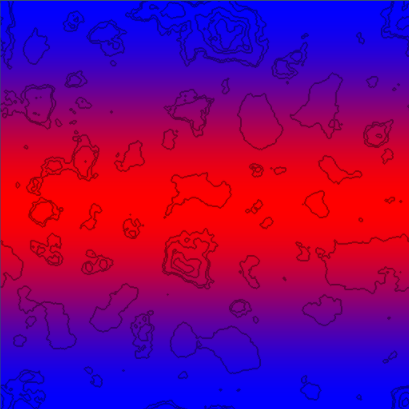This data is a great start, as we want to have a warm band in the center of the map, similar to the equator on earth. This will the the base heat map we will build off of.

The next thing we want to do, is define HeatType zones, similar to how we defined HeightType zones in the previous part of this tutorial.

```public enum HeatType
{
Coldest,
Colder,
Cold,
Warm,
Warmer,
Warmest
}
```

These HeatTypes will be adjustable from the Unity inspector, with the help of a few new variables:

```	float ColdestValue = 0.05f;
float ColderValue = 0.18f;
float ColdValue = 0.4f;
float WarmValue = 0.6f;
float WarmerValue = 0.8f;
```

In LoadTiles, we set the HeatType of each tile, based on its heat value.

```// set heat type
if (heatValue < ColdestValue)
t.HeatType = HeatType.Coldest;
else if (heatValue < ColderValue)
t.HeatType = HeatType.Colder;
else if (heatValue < ColdValue)
t.HeatType = HeatType.Cold;
else if (heatValue < WarmValue)
t.HeatType = HeatType.Warm;
else if (heatValue < WarmerValue)
t.HeatType = HeatType.Warmer;
else
t.HeatType = HeatType.Warmest;
```

Finally, we will add some new colors, for each HeatType in our TextureGenerator class:

```	// Height Map Colors
private static Color Coldest = new Color(0, 1, 1, 1);
private static Color Colder = new Color(170/255f, 1, 1, 1);
private static Color Cold = new Color(0, 229/255f, 133/255f, 1);
private static Color Warm = new Color(1, 1, 100/255f, 1);
private static Color Warmer = new Color(1, 100/255f, 0, 1);
private static Color Warmest = new Color(241/255f, 12/255f, 0, 1);

public static Texture2D GetHeatMapTexture(int width, int height, Tile[,] tiles)
{
var texture = new Texture2D(width, height);
var pixels = new Color[width * height];

for (var x = 0; x < width; x++)
{
for (var y = 0; y < height; y++)
{
switch (tiles[x,y].HeatType)
{
case HeatType.Coldest:
pixels[x + y * width] = Coldest;
break;
case HeatType.Colder:
pixels[x + y * width] = Colder;
break;
case HeatType.Cold:
pixels[x + y * width] = Cold;
break;
case HeatType.Warm:
pixels[x + y * width] = Warm;
break;
case HeatType.Warmer:
pixels[x + y * width] = Warmer;
break;
case HeatType.Warmest:
pixels[x + y * width] = Warmest;
break;
}

//darken the color if a edge tile
pixels[x + y * width] = Color.Lerp(pixels[x + y * width], Color.black, 0.4f);
}
}

texture.SetPixels(pixels);
texture.wrapMode = TextureWrapMode.Clamp;
texture.Apply();
return texture;
}
```

Generating this heat texture, now gives us the following: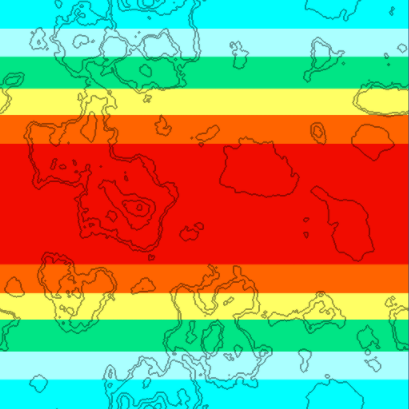We can now clearly see our defined HeatType zones. This data, however, is still just bands. It doesn't provide us with anything but latitude based heat data. Since temperature in the real world is reliant on a multitude of factors, we are going to blend in some fractal noise with this gradient noise.

We will add a couple of new variables to our Generator, and a new Fractal:

```
int HeatOctaves = 4;
double HeatFrequency = 3.0;

private void Initialize()
{
// Initialize the Heat map
ImplicitGradient gradient  = new ImplicitGradient (1, 1, 1, 0, 1, 1, 1, 1, 1, 1, 1, 1);
ImplicitFractal heatFractal = new ImplicitFractal(FractalType.MULTI,
BasisType.SIMPLEX,
InterpolationType.QUINTIC,
HeatOctaves,
HeatFrequency,
Seed);

// Combine the gradient with our heat fractal
HeatMap = new ImplicitCombiner (CombinerType.MULTIPLY);
}
```

By combining the fractal with the gradient using a Multiply operation, the resulting noise gets multiplied based on the latitude. The Multiply operation is illustrated below: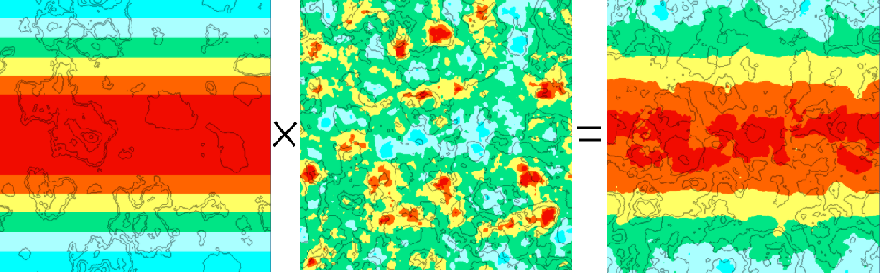Gradient noise on the left, fractal noise in the middle, result of the Multiply operation on the right. As you can see, we now have a much nicer, less bandy heat map.

That takes care of the latitude portion. Next, we need to take the height map into consideration. We want our tallest mountaintops to be cold. The adjustment can easily be done in the LoadTiles function:

```// Adjust Heat Map based on Height - Higher == colder
if (t.HeightType == HeightType.Grass) {
HeatData.Data[t.X, t.Y] -= 0.1f * t.HeightValue;
}
else if (t.HeightType == HeightType.Forest) {
HeatData.Data[t.X, t.Y] -= 0.2f * t.HeightValue;
}
else if (t.HeightType == HeightType.Rock) {
HeatData.Data[t.X, t.Y] -= 0.3f * t.HeightValue;
}
else if (t.HeightType == HeightType.Snow) {
HeatData.Data[t.X, t.Y] -= 0.4f * t.HeightValue;
}
```

This adjustment gives us our final Heat Map, taking both latitude and height into consideration: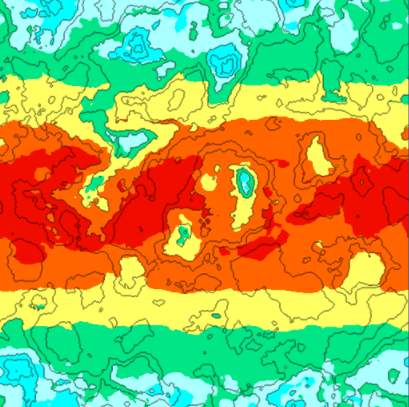### Generating the Moisture Map

The moisture map is similar to the heat map. We first generate a fractal to give us a random base. Then we adjust this data based on the height map.

We will go through the moisture code quickly, as it is very similar to the heat map code.

First, the Tile class is going to need a new MoistureType

```public enum MoistureType
{
Wettest,
Wetter,
Wet,
Dry,
Dryer,
Dryest
}
```

The Generator class will need new exposed variables, for the Unity Inspector:

```	int MoistureOctaves = 4;
double MoistureFrequency = 3.0;
float DryerValue = 0.27f;
float DryValue = 0.4f;
float WetValue = 0.6f;
float WetterValue = 0.8f;
float WettestValue = 0.9f;
```

The TextureGenerator is going to need a new MoistureMap generation function, and associated colors:

```	//Moisture map
private static Color Dryest = new Color(255/255f, 139/255f, 17/255f, 1);
private static Color Dryer = new Color(245/255f, 245/255f, 23/255f, 1);
private static Color Dry = new Color(80/255f, 255/255f, 0/255f, 1);
private static Color Wet = new Color(85/255f, 255/255f, 255/255f, 1);
private static Color Wetter = new Color(20/255f, 70/255f, 255/255f, 1);
private static Color Wettest = new Color(0/255f, 0/255f, 100/255f, 1);
```

```	public static Texture2D GetMoistureMapTexture(int width, int height, Tile[,] tiles)
{
var texture = new Texture2D(width, height);
var pixels = new Color[width * height];

for (var x = 0; x < width; x++)
{
for (var y = 0; y < height; y++)
{
Tile t = tiles[x,y];

if (t.MoistureType == MoistureType.Dryest)
pixels[x + y * width] = Dryest;
else if (t.MoistureType == MoistureType.Dryer)
pixels[x + y * width] = Dryer;
else if (t.MoistureType == MoistureType.Dry)
pixels[x + y * width] = Dry;
else if (t.MoistureType == MoistureType.Wet)
pixels[x + y * width] = Wet;
else if (t.MoistureType == MoistureType.Wetter)
pixels[x + y * width] = Wetter;
else
pixels[x + y * width] = Wettest;
}
}

texture.SetPixels(pixels);
texture.wrapMode = TextureWrapMode.Clamp;
texture.Apply();
return texture;
}

```

Finally, our LoadTiles function will set a MoistureType based on its MoistureValue:

```//Moisture Map Analyze
float moistureValue = MoistureData.Data[x,y];
moistureValue = (moistureValue - MoistureData.Min) / (MoistureData.Max - MoistureData.Min);
t.MoistureValue = moistureValue;

//set moisture type
if (moistureValue < DryerValue) t.MoistureType = MoistureType.Dryest;
else if (moistureValue < DryValue) t.MoistureType = MoistureType.Dryer;
else if (moistureValue < WetValue) t.MoistureType = MoistureType.Dry;
else if (moistureValue < WetterValue) t.MoistureType = MoistureType.Wet;
else if (moistureValue < WettestValue) t.MoistureType = MoistureType.Wetter;
else t.MoistureType = MoistureType.Wettest;
```

Rendering the initial noise for the MoistureMap, gives us the following: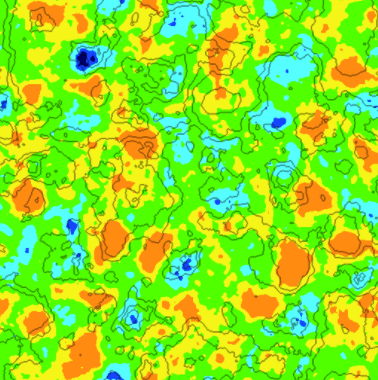The only thing left to do, is adjust the moisture map according to height map. We make the adjustment in the LoadTiles function:

```//adjust moisture based on height
if (t.HeightType == HeightType.DeepWater) {
MoistureData.Data[t.X, t.Y] += 8f * t.HeightValue;
}
else if (t.HeightType == HeightType.ShallowWater) {
MoistureData.Data[t.X, t.Y] += 3f * t.HeightValue;
}
else if (t.HeightType == HeightType.Shore) {
MoistureData.Data[t.X, t.Y] += 1f * t.HeightValue;
}
else if (t.HeightType == HeightType.Sand) {
MoistureData.Data[t.X, t.Y] += 0.25f * t.HeightValue;
}

```

Since we now adjusted our moisture data based on the height of certain tiles, our updated moisture map looks a lot nicer: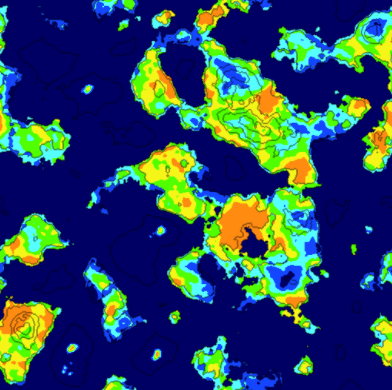### Generating Rivers

The river generation method I will describe is really just a brute force attempt by my part, to make convincing looking rivers.

The first step of the algorithm, is to select a random tile on the map. The selected tile must be land, and must also have a height value that is over a specified threshold.

From this tile, we determine which neighboring tile is the lowest, and navigate towards it. We create a path in this fashion, until a water tile is reached.

If the generated path meets our criterias (river length, number of turns, number of intersections), we save the path for later use.

Otherwise, we discard the path, and try again. The following code gets us started:

```private void GenerateRivers()
{
int attempts = 0;
int rivercount = RiverCount;
Rivers = new List<River> ();

// Generate some rivers
while (rivercount > 0 && attempts < MaxRiverAttempts) {

// Get a random tile
int x = UnityEngine.Random.Range (0, Width);
int y = UnityEngine.Random.Range (0, Height);
Tile tile = Tiles[x,y];

// validate the tile
if (!tile.Collidable) continue;
if (tile.Rivers.Count > 0) continue;

if (tile.HeightValue > MinRiverHeight)
{
// Tile is good to start river from
River river = new River(rivercount);

// Figure out the direction this river will try to flow
river.CurrentDirection = tile.GetLowestNeighbor ();

// Recursively find a path to water
FindPathToWater(tile, river.CurrentDirection, ref river);

// Validate the generated river
if (river.TurnCount < MinRiverTurns || river.Tiles.Count < MinRiverLength || river.Intersections > MaxRiverIntersections)
{
//Validation failed - remove this river
for (int i = 0; i < river.Tiles.Count; i++)
{
Tile t = river.Tiles[i];
t.Rivers.Remove (river);
}
}
else if (river.Tiles.Count >= MinRiverLength)
{
//Validation passed - Add river to list
rivercount--;
}
}
attempts++;
}
}

```

The recursive FindPathToWater() function, determines the best path to take based on the terrain height, existing rivers, and its preferred direction. It will eventually find a water tile. We recursively call the function until the path is complete.

```private void FindPathToWater(Tile tile, Direction direction, ref River river)
{
if (tile.Rivers.Contains (river))
return;

// check if there is already a river on this tile
if (tile.Rivers.Count > 0)
river.Intersections++;

// get neighbors
Tile left = GetLeft (tile);
Tile right = GetRight (tile);
Tile top = GetTop (tile);
Tile bottom = GetBottom (tile);

float leftValue = int.MaxValue;
float rightValue = int.MaxValue;
float topValue = int.MaxValue;
float bottomValue = int.MaxValue;

// query height values of neighbors
if (left.GetRiverNeighborCount(river) < 2 && !river.Tiles.Contains(left))
leftValue = left.HeightValue;
if (right.GetRiverNeighborCount(river) < 2 && !river.Tiles.Contains(right))
rightValue = right.HeightValue;
if (top.GetRiverNeighborCount(river) < 2 && !river.Tiles.Contains(top))
topValue = top.HeightValue;
if (bottom.GetRiverNeighborCount(river) < 2 && !river.Tiles.Contains(bottom))
bottomValue = bottom.HeightValue;

// if neighbor is existing river that is not this one, flow into it
if (bottom.Rivers.Count == 0 && !bottom.Collidable)
bottomValue = 0;
if (top.Rivers.Count == 0 && !top.Collidable)
topValue = 0;
if (left.Rivers.Count == 0 && !left.Collidable)
leftValue = 0;
if (right.Rivers.Count == 0 && !right.Collidable)
rightValue = 0;

// override flow direction if a tile is significantly lower
if (direction == Direction.Left)
if (Mathf.Abs (rightValue - leftValue) < 0.1f)
rightValue = int.MaxValue;
if (direction == Direction.Right)
if (Mathf.Abs (rightValue - leftValue) < 0.1f)
leftValue = int.MaxValue;
if (direction == Direction.Top)
if (Mathf.Abs (topValue - bottomValue) < 0.1f)
bottomValue = int.MaxValue;
if (direction == Direction.Bottom)
if (Mathf.Abs (topValue - bottomValue) < 0.1f)
topValue = int.MaxValue;

// find mininum
float min = Mathf.Min (Mathf.Min (Mathf.Min (leftValue, rightValue), topValue), bottomValue);

// if no minimum found - exit
if (min == int.MaxValue)
return;

//Move to next neighbor
if (min == leftValue) {
if (left.Collidable)
{
if (river.CurrentDirection != Direction.Left){
river.TurnCount++;
river.CurrentDirection = Direction.Left;
}
FindPathToWater (left, direction, ref river);
}
} else if (min == rightValue) {
if (right.Collidable)
{
if (river.CurrentDirection != Direction.Right){
river.TurnCount++;
river.CurrentDirection = Direction.Right;
}
FindPathToWater (right, direction, ref river);
}
} else if (min == bottomValue) {
if (bottom.Collidable)
{
if (river.CurrentDirection != Direction.Bottom){
river.TurnCount++;
river.CurrentDirection = Direction.Bottom;
}
FindPathToWater (bottom, direction, ref river);
}
} else if (min == topValue) {
if (top.Collidable)
{
if (river.CurrentDirection != Direction.Top){
river.TurnCount++;
river.CurrentDirection = Direction.Top;
}
FindPathToWater (top, direction, ref river);
}
}
}
```

After running this river path generation process, we are left with a bunch of paths that lead to water. This resembles the following: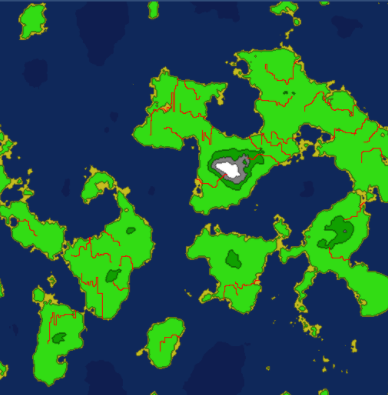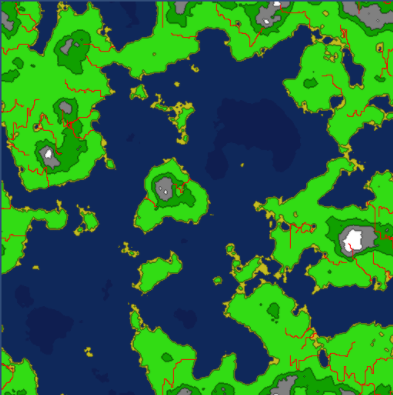A lot of the paths intersect, and if we were to dig these rivers out now, they might look a little strange if their sizes didn't match up at the point of intersection. Because of this, we are going to need to determine which rivers are intersecting, and group them together.

We will need a RiverGroup class:

```public class RiverGroup
{
public List<River> Rivers = new List<River>();
}
```

And the code to group the river paths together, if they intersect:

```private void BuildRiverGroups()
{
//loop each tile, checking if it belongs to multiple rivers
for (var x = 0; x < Width; x++) {
for (var y = 0; y < Height; y++) {
Tile t = Tiles[x,y];

if (t.Rivers.Count > 1)
{
// multiple rivers == intersection
RiverGroup group = null;

// Does a rivergroup already exist for this group?
for (int n=0; n < t.Rivers.Count; n++)
{
River tileriver = t.Rivers[n];
for (int i = 0; i < RiverGroups.Count; i++)
{
for (int j = 0; j < RiverGroups[i].Rivers.Count; j++)
{
River river = RiverGroups[i].Rivers[j];
if (river.ID == tileriver.ID)
{
group = RiverGroups[i];
}
if (group != null) break;
}
if (group != null) break;
}
if (group != null) break;
}

// existing group found -- add to it
if (group != null)
{
for (int n=0; n < t.Rivers.Count; n++)
{
if (!group.Rivers.Contains(t.Rivers[n]))
}
}
else   //No existing group found - create a new one
{
group = new RiverGroup();
for (int n=0; n < t.Rivers.Count; n++)
{
}
}
}
}
}
}
```

Now, we have a groups of rivers, that intersect, leading to water. Rendering these groups of rivers looks like the following, each group represented by a random color: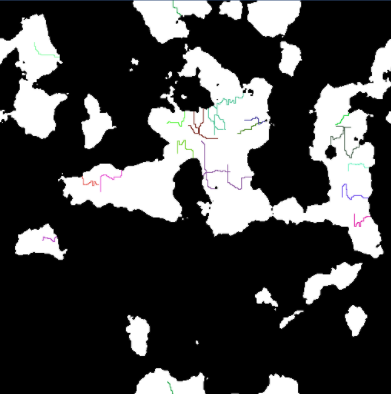With this information, we can start digging out the rivers. For each river group, we first start by digging out the longest river in the group. The remaining rivers in the group are dug out based off this longest route.

The following code shows us how we start digging out the river groups:

```private void DigRiverGroups()
{
for (int i = 0; i < RiverGroups.Count; i++) {

RiverGroup group = RiverGroups[i];
River longest = null;

//Find longest river in this group
for (int j = 0; j < group.Rivers.Count; j++)
{
River river = group.Rivers[j];
if (longest == null)
longest = river;
else if (longest.Tiles.Count < river.Tiles.Count)
longest = river;
}

if (longest != null)
{
//Dig out longest path first
DigRiver (longest);

for (int j = 0; j < group.Rivers.Count; j++)
{
River river = group.Rivers[j];
if (river != longest)
{
DigRiver (river, longest);
}
}
}
}
}
```

The code to dig out a river is a little more complicated, as it attempts to randomize as many parameters as possible.

It is also important for the rivers to widen as it approaches water. The DigRiver() code isn't pretty, but it does its job:

```private void DigRiver(River river)
{
int counter = 0;

// How wide are we digging this river?
int size = UnityEngine.Random.Range(1,5);
river.Length = river.Tiles.Count;

// randomize size change
int two = river.Length / 2;
int three = two / 2;
int four = three / 2;
int five = four / 2;

int twomin = two / 3;
int threemin = three / 3;
int fourmin = four / 3;
int fivemin = five / 3;

// randomize lenght of each size
int count1 = UnityEngine.Random.Range (fivemin, five);
if (size < 4) {
count1 = 0;
}
int count2 = count1 + UnityEngine.Random.Range(fourmin, four);
if (size < 3) {
count2 = 0;
count1 = 0;
}
int count3 = count2 + UnityEngine.Random.Range(threemin, three);
if (size < 2) {
count3 = 0;
count2 = 0;
count1 = 0;
}
int count4 = count3 + UnityEngine.Random.Range (twomin, two);

// Make sure we are not digging past the river path
if (count4 > river.Length) {
int extra = count4 - river.Length;
while (extra > 0)
{
if (count1 > 0) { count1--; count2--; count3--; count4--; extra--; }
else if (count2 > 0) { count2--; count3--; count4--; extra--; }
else if (count3 > 0) { count3--; count4--; extra--; }
else if (count4 > 0) { count4--; extra--; }
}
}

// Dig it out
for (int i = river.Tiles.Count - 1; i >= 0 ; i--)
{
Tile t = river.Tiles[i];

if (counter < count1) {
t.DigRiver (river, 4);
}
else if (counter < count2) {
t.DigRiver (river, 3);
}
else if (counter < count3) {
t.DigRiver (river, 2);
}
else if ( counter < count4) {
t.DigRiver (river, 1);
}
else {
t.DigRiver(river, 0);
}
counter++;
}
}

```

Digging out these rivers, provides us with something that looks like this: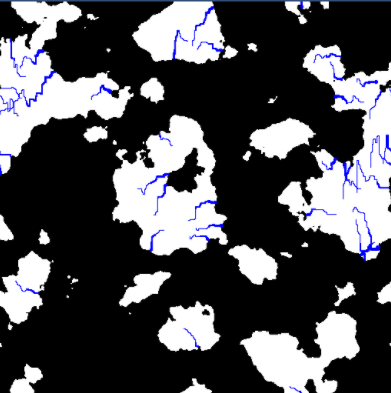This provides us with some somewhat convincing rivers, however, we still need to make sure they provide moisture to our map. The rivers would not appear in a desert area, therefore, we need to make sure that the area around the rivers are not dry.

To facilitate this process, we add a new function, to adjust the moisture map based on our river data.

```private void AdjustMoistureMap()
{
for (var x = 0; x < Width; x++) {
for (var y = 0; y < Height; y++) {

Tile t = Tiles[x,y];
if (t.HeightType == HeightType.River)
{
}
}
}
}
```

The moisture that is added varies, based on the distance of the originating tile. The further away from the river, the less moisture a tile will receive.

```
{
int startx = MathHelper.Mod (t.X - radius, Width);
int endx = MathHelper.Mod (t.X + radius, Width);
Vector2 center = new Vector2(t.X, t.Y);

while (curr > 0) {

int x1 = MathHelper.Mod (t.X - curr, Width);
int x2 = MathHelper.Mod (t.X + curr, Width);
int y = t.Y;

AddMoisture(Tiles[x1, y], 0.025f / (center - new Vector2(x1, y)).magnitude);

for (int i = 0; i < curr; i++)
{
AddMoisture (Tiles[x1, MathHelper.Mod (y + i + 1, Height)], 0.025f / (center - new Vector2(x1, MathHelper.Mod (y + i + 1, Height))).magnitude);
AddMoisture (Tiles[x1, MathHelper.Mod (y - (i + 1), Height)], 0.025f / (center - new Vector2(x1, MathHelper.Mod (y - (i + 1), Height))).magnitude);

AddMoisture (Tiles[x2, MathHelper.Mod (y + i + 1, Height)], 0.025f / (center - new Vector2(x2, MathHelper.Mod (y + i + 1, Height))).magnitude);
AddMoisture (Tiles[x2, MathHelper.Mod (y - (i + 1), Height)], 0.025f / (center - new Vector2(x2, MathHelper.Mod (y - (i + 1), Height))).magnitude);
}
curr--;
}
}

```

Making this adjustment provides us with an updated moisture map, that takes the rivers into account. This will come in handy in the next section, when we start generating biomes.

The updated moisture map, looks like the following: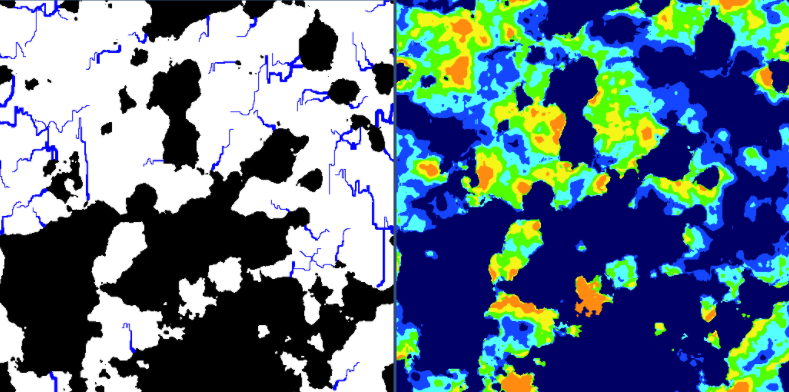Stay tuned for Part 4. It will be the best part, as we are going to put all of these maps together and make a real world.

Source Code for Part 3 on github.

Continue to Part 4 of this series.

## Procedurally Generating Wrapping World Maps in Unity C# – Part 2

Check out Part 1 of this series if you haven’t already. This is a continuation of that article.

In the previous article (Part 1):

In Part 3:

In Part 4:

### Wrapping the Map on One Axis

In part 1 of this tutorial, we set up a nice little framework to help us build up our maps. The Height Map we created previously, was not tileable.

This is because we sampled 2D noise data, which is not capable of providing us with what we need. If we want to make our world wrap around seamlessly, then we are going to need to add some dimension to our noise generator.

With 3D noise, we can sample data in a circular pattern, and the resulting 2D data will wrap on a single axis. The sampled data would resemble a cylinder in 3D space.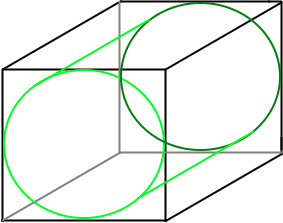Imagine if we took this cylinder, cut it open, and laid it flat. This is essentially what we will be doing. The ends where we made the cut, would be able to join together seamlessly.

In order to do this, we need to modify the GetData function in our Generator class.

```private void GetData(ImplicitModuleBase module, ref MapData mapData)
{
mapData = new MapData (Width, Height);

// loop through each x,y point - get height value
for (var x = 0; x < Width; x++) {
for (var y = 0; y < Height; y++) {

//Noise range
float x1 = 0, x2 = 1;
float y1 = 0, y2 = 1;
float dx = x2 - x1;
float dy = y2 - y1;

//Sample noise at smaller intervals
float s = x / (float)Width;
float t = y / (float)Height;

// Calculate our 3D coordinates
float nx = x1 + Mathf.Cos (s * 2 * Mathf.PI) * dx / (2 * Mathf.PI);
float ny = x1 + Mathf.Sin (s * 2 * Mathf.PI) * dx / (2 * Mathf.PI);
float nz = t;

float heightValue = (float)HeightMap.Get (nx, ny, nz);

// keep track of the max and min values found
if (heightValue > mapData.Max)
mapData.Max = heightValue;
if (heightValue < mapData.Min)
mapData.Min = heightValue;

mapData.Data [x, y] = heightValue;
}
}
}
```

Running this code, then gives us a nice texture, that wraps on the x-axis: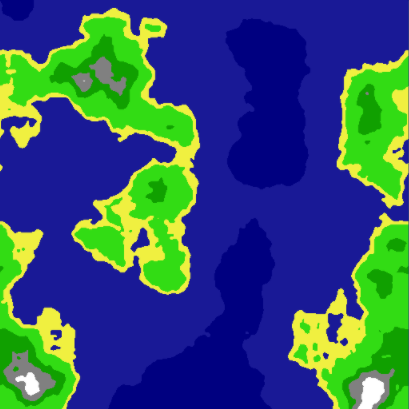### Wrapping the Map on Both Axis

In order to get our map to wrap around both axis, we need to start sampling 4D noise. This concept is a little more difficult to grasp, as our minds have a hard time thinking in 4 dimensions, but is very similar to the 3D example.

Instead of having a single cylinder, you would have two cylinders connected together, in a 4D space.

Keep in mind, that sampling 4D data takes a lot longer than sampling 2D data.

Our updated GetData() function would then look like this:

```private void GetData(ImplicitModuleBase module, ref MapData mapData)
{
mapData = new MapData (Width, Height);

// loop through each x,y point - get height value
for (var x = 0; x < Width; x++) {
for (var y = 0; y < Height; y++) {

// Noise range
float x1 = 0, x2 = 2;
float y1 = 0, y2 = 2;
float dx = x2 - x1;
float dy = y2 - y1;

// Sample noise at smaller intervals
float s = x / (float)Width;
float t = y / (float)Height;

// Calculate our 4D coordinates
float nx = x1 + Mathf.Cos (s*2*Mathf.PI) * dx/(2*Mathf.PI);
float ny = y1 + Mathf.Cos (t*2*Mathf.PI) * dy/(2*Mathf.PI);
float nz = x1 + Mathf.Sin (s*2*Mathf.PI) * dx/(2*Mathf.PI);
float nw = y1 + Mathf.Sin (t*2*Mathf.PI) * dy/(2*Mathf.PI);

float heightValue = (float)HeightMap.Get (nx, ny, nz, nw);

// keep track of the max and min values found
if (heightValue > mapData.Max) mapData.Max = heightValue;
if (heightValue < mapData.Min) mapData.Min = heightValue;

mapData.Data[x,y] = heightValue;
}
}
}
```

This code produces a seamless tileable texture, that is procedurally generated from 4D noise: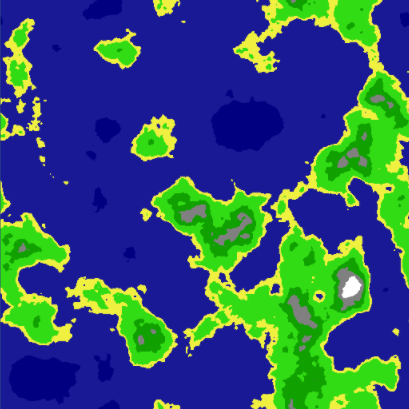If you would like more information on how this works, have a look here and here.

### Finding Neighbors

Now that we have a tileable Height Map, we are starting to get a lot closer to our goal. Now, we are going to shift focus towards the Tile class.

It would be very useful if each Tile object had a reference to each of its neighbors (top, bottom, left, right). This comes in handy for things, such as creating paths, bitmasking, or flood filling. We will touch on these aspects later on in this tutorial.

First thing we need to do, is create variables in our Tile class:

```public Tile Left;
public Tile Right;
public Tile Top;
public Tile Bottom;
```

The next part is pretty straightforward. We simply run through every single tile, setting it’s neighboring tiles. First off, we will create a few functions inside of our Generator class, to simplify retrieving the Tile neighbors:

```	private Tile GetTop(Tile t)
{
return Tiles [t.X, MathHelper.Mod (t.Y - 1, Height)];
}
private Tile GetBottom(Tile t)
{
return Tiles [t.X, MathHelper.Mod (t.Y + 1, Height)];
}
private Tile GetLeft(Tile t)
{
return Tiles [MathHelper.Mod(t.X - 1, Width), t.Y];
}
private Tile GetRight(Tile t)
{
return Tiles [MathHelper.Mod (t.X + 1, Width), t.Y];
}
```

MathHelper.Mod() will wrap the x and y values for us, based on our Map width and height. This will ensure we never go off of our map.

Next, we also need to add the function that will do the neighbor assignments:

```	private void UpdateNeighbors()
{
for (var x = 0; x < Width; x++)
{
for (var y = 0; y < Height; y++)
{
Tile t = Tiles[x,y];

t.Top = GetTop(t);
t.Bottom = GetBottom (t);
t.Left = GetLeft (t);
t.Right = GetRight (t);
}
}
}
```

Visually, this doesn’t do much, yet. However, each Tile now knows who their neighbors are, which is very important for future steps.

I decided to add this into the tutorial, mainly for aesthetic purposes. Bitmasking, in this context, is about assigning a value to each tile, based on it’s neighbors. Have a look at the following image: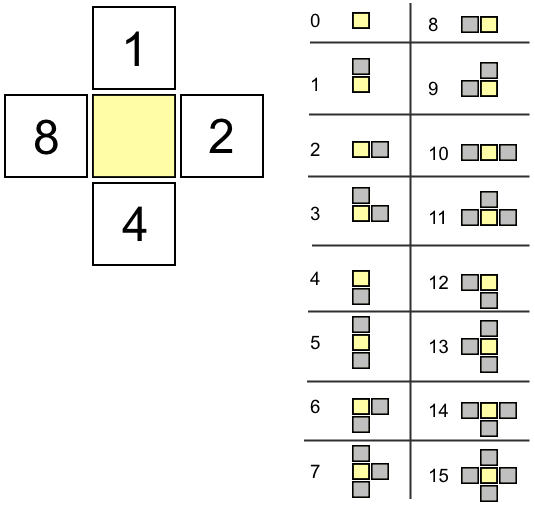Based on a tile’s neighbors, we increment the bitmask as shown on the left side of the above image. All possibilities are illustrated on the right side. Note that each value is unique. This allows us to identify a block’s configuration very quickly.

The main benefit of bitmasking, is that you can then assign a texture, based on the bitmask value of each tile, making your maps a lot prettier and way less blocky when done properly.

Another benefit of bitmasking, is that if a Tile’s bitmask value is not equal to 15, then we know it is an edge tile.

Let’s add a function in our Tile class to do the calculation. We are only concerned with neighbors that share the same Height Type as the tile being analyzed.

```	public void UpdateBitmask()
{
int count = 0;

if (Top.HeightType == HeightType)
count += 1;
if (Right.HeightType == HeightType)
count += 2;
if (Bottom.HeightType == HeightType)
count += 4;
if (Left.HeightType == HeightType)
count += 8;

}
```

Since we already have references to the neighboring tiles, and we also have defined a HeightType, this calculation is quite trivial. Next, we add a function in our Generator class, in order to process this calculation for all of the tiles:

```	private void UpdateBitmasks()
{
for (var x = 0; x < Width; x++) {
for (var y = 0; y < Height; y++) {
}
}
}
```

Now, if we modify our TextureGenerator as follows:

```//darken the color if a edge tile
pixels[x + y * width] = Color.Lerp(pixels[x + y * width], Color.black, 0.4f);

```

We can now see a defined edge between our Height Types: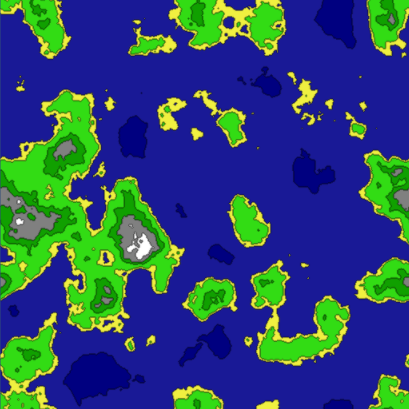### Flood Filling

It would be nice if we could determine a few things, such as:

• Where are the Lakes?
• Where are the Oceans?
• Where are the Land Masses?
• How big are each of these?

We can answer all of these question, with the help of a simple Flood Fill algorithm.

First, we are going to create an object, that will store information on our Tiles:

```using UnityEngine;
using System.Collections.Generic;

public enum TileGroupType
{
Water,
Land
}

public class TileGroup  {

public TileGroupType Type;
public List<Tile> Tiles;

public TileGroup()
{
Tiles = new List<Tile> ();
}
}
```

The TileGroup class will hold a reference to a list of Tiles. It will also let us know if this particular group is Water or Land.

The main idea is to break down connected pieces of land and water into TileGroup collections.

We are also going to modify the Tile class slightly by adding two new variables:

```	public bool Collidable;
public bool FloodFilled;
```

Collidable will be set inside of the LoadTiles() method. Anything that is not a water tile, will have Collidable set to true. The FloodFilled variable will be used to keep track of which tiles have already been processed by the flood filling algorithm.

In order to add our flood fill algorithm to the Generator class. First we are going to need a couple of TileGroup variables:

```	List<TileGroup> Waters = new List<TileGroup> ();
List<TileGroup> Lands = new List<TileGroup> ();
```

Now we are ready to determine land and water masses in our map.

Since the map could potentially be very large, we cannot use a recursive flood fill, as it would easily produce stack overflow exceptions. Instead, we will need to use a non-recursive approach to solve this problem:

```
private void FloodFill()
{
// Use a stack instead of recursion
Stack<Tile> stack = new Stack<Tile>();

for (int x = 0; x < Width; x++) {
for (int y = 0; y < Height; y++) {

Tile t = Tiles[x,y];

if (t.FloodFilled) continue;

// Land
if (t.Collidable)
{
TileGroup group = new TileGroup();
group.Type = TileGroupType.Land;
stack.Push(t);

while(stack.Count > 0) {
FloodFill(stack.Pop(), ref group, ref stack);
}

if (group.Tiles.Count > 0)
}
// Water
else {
TileGroup group = new TileGroup();
group.Type = TileGroupType.Water;
stack.Push(t);

while(stack.Count > 0)	{
FloodFill(stack.Pop(), ref group, ref stack);
}

if (group.Tiles.Count > 0)
}
}
}
}

private void FloodFill(Tile tile, ref TileGroup tiles, ref Stack<Tile> stack)
{
// Validate
if (tile.FloodFilled)
return;
if (tiles.Type == TileGroupType.Land && !tile.Collidable)
return;
if (tiles.Type == TileGroupType.Water && tile.Collidable)
return;

tile.FloodFilled = true;

// floodfill into neighbors
Tile t = GetTop (tile);
if (!t.FloodFilled && tile.Collidable == t.Collidable)
stack.Push (t);
t = GetBottom (tile);
if (!t.FloodFilled && tile.Collidable == t.Collidable)
stack.Push (t);
t = GetLeft (tile);
if (!t.FloodFilled && tile.Collidable == t.Collidable)
stack.Push (t);
t = GetRight (tile);
if (!t.FloodFilled && tile.Collidable == t.Collidable)
stack.Push (t);
}

```

Using the above code, will separate all land and water masses and put them into TileGroups

I generated a couple of textures to demonstrate how useful this data can be.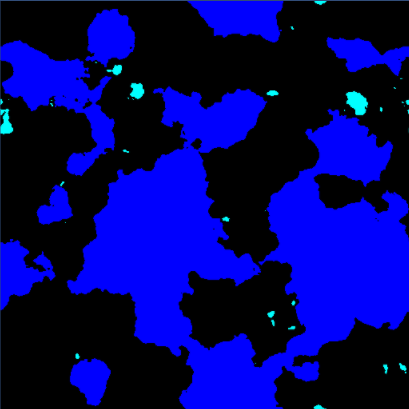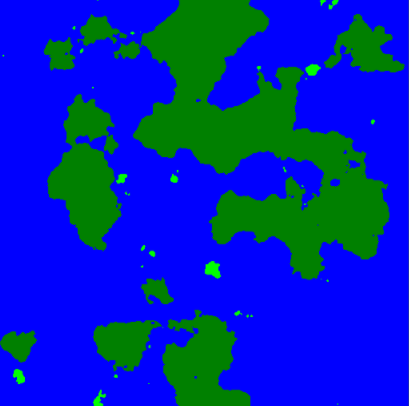The left side image, all land tiles are all black. The ocean tiles are blue, and the lake tiles are cyan.

The right side image, all water tiles are blue. Large land masses are dark green, and islands in light green.

As you can see, we now have a lot more information on our generated map, and it effectively answers all of the questions we set out to answer.

Source code for Part 2 can be found here on github.

Continue to Part 3 of this series.

## Procedurally Generating Wrapping World Maps in Unity C# – Part 1

In Part 2:

In Part 3:

In Part 4:

### Introduction

I always like to start these tutorials with an example of what the final output will resemble: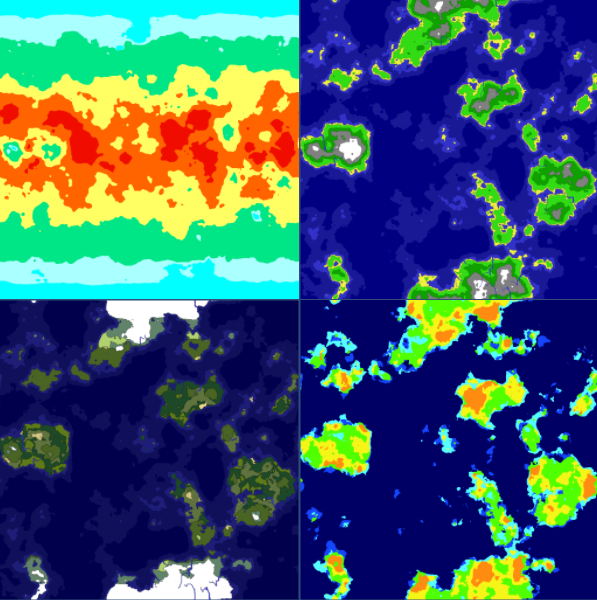The map representations you see above are:

• Heat Map (Top Left)
• Height Map (Top Right)
• Moisture Map (Bottom Right)
• Biome Map (Bottom Left)

These are the kind of maps I will be showing you how to create, with these tutorials

### Noise Generation

There are a multitude of different noise generators on the internet, most of which are open sourced. There is no need to re-invent the wheel here, so I opted to use a custom port of the Accidental Noise library.

The C# port was done by Nikolaj Mariager.

Some minor adjustments were made to his port in order to get it to work properly in Unity.

### Getting Started

First, we need some kind of container to store the data that we are going to generate.

So, let’s start off by creating a MapData class. The Min and Max variables will serve as a way to keep track of our generated upper and lower limits.

```public class MapData {

public float[,] Data;
public float Min { get; set; }
public float Max { get; set; }

public MapData(int width, int height)
{
Data = new float[width, height];
Min = float.MaxValue;
Max = float.MinValue;
}
}
```

We are also going to create a Tile class, which will be used to eventually create our Unity gameobjects, from our generated data.

```public class Tile
{
public float HeightValue { get; set; }
public int X, Y;

public Tile()
{
}
}
```

In order to see what is going on, we will need some sort of visual representation of the data. For this we create a new TextureGenerator class.

For the time being, this class will simply generate a black and white representation of our data.

```using UnityEngine;

public static class TextureGenerator {

public static Texture2D GetTexture(int width, int height, Tile[,] tiles)
{
var texture = new Texture2D(width, height);
var pixels = new Color[width * height];

for (var x = 0; x < width; x++)
{
for (var y = 0; y < height; y++)
{
float value = tiles[x, y].HeightValue;

//Set color range, 0 = black, 1 = white
pixels[x + y * width] = Color.Lerp (Color.black, Color.white, value);
}
}

texture.SetPixels(pixels);
texture.wrapMode = TextureWrapMode.Clamp;
texture.Apply();
return texture;
}

}
```

We will expand on this Texture Generator soon.

### Generating the Height Map

Since I decided that the maps are going to be fixed size, we need to set a map Width and Height. We also need a few adjustable parameters for the noise generator.

We are going to expose these variables to the Unity Inspector, as it will make tuning the maps a lot easier.

The Generator class initializes the Noise module, generates height map data, creates an array of tiles, then generates a texture representation of this data.

Have a look at the code, along with the comments:

```using UnityEngine;
using AccidentalNoise;

public class Generator : MonoBehaviour {

// Adjustable variables for Unity Inspector
[SerializeField]
int Width = 256;
[SerializeField]
int Height = 256;
[SerializeField]
int TerrainOctaves = 6;
[SerializeField]
double TerrainFrequency = 1.25;

// Noise generator module
ImplicitFractal HeightMap;

// Height map data
MapData HeightData;

// Final Objects
Tile[,] Tiles;

// Our texture output (unity component)
MeshRenderer HeightMapRenderer;

void Start()
{
// Get the mesh we are rendering our output to
HeightMapRenderer = transform.Find ("HeightTexture").GetComponent<MeshRenderer> ();

// Initialize the generator
Initialize ();

// Build the height map
GetData (HeightMap, ref HeightData);

// Build our final objects based on our data

// Render a texture representation of our map
HeightMapRenderer.materials.mainTexture = TextureGenerator.GetTexture (Width, Height, Tiles);
}

private void Initialize()
{
// Initialize the HeightMap Generator
HeightMap = new ImplicitFractal (FractalType.MULTI,
BasisType.SIMPLEX,
InterpolationType.QUINTIC,
TerrainOctaves,
TerrainFrequency,
UnityEngine.Random.Range (0, int.MaxValue));
}

// Extract data from a noise module
private void GetData(ImplicitModuleBase module, ref MapData mapData)
{
mapData = new MapData (Width, Height);

// loop through each x,y point - get height value
for (var x = 0; x < Width; x++)
{
for (var y = 0; y < Height; y++)
{
//Sample the noise at smaller intervals
float x1 = x / (float)Width;
float y1 = y / (float)Height;

float value = (float)HeightMap.Get (x1, y1);

//keep track of the max and min values found
if (value > mapData.Max) mapData.Max = value;
if (value < mapData.Min) mapData.Min = value;

mapData.Data[x,y] = value;
}
}
}

// Build a Tile array from our data
{
Tiles = new Tile[Width, Height];

for (var x = 0; x < Width; x++)
{
for (var y = 0; y < Height; y++)
{
Tile t = new Tile();
t.X = x;
t.Y = y;

float value = HeightData.Data[x, y];

//normalize our value between 0 and 1
value = (value - HeightData.Min) / (HeightData.Max - HeightData.Min);

t.HeightValue = value;

Tiles[x,y] = t;
}
}
}

}
```
Now, if we run this code, we get the following output texture: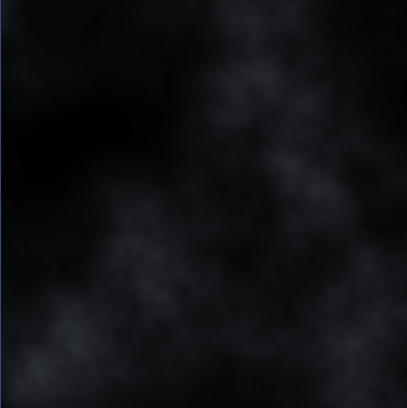Doesn't look like much yet, however, it is a very good start. We have an array of data, containing values between 0 and 1, with some very interesting patterns.

Now, we need to start assigning some meaning to this data. For example, we can say that anything that is less than 0.4 is considered water.

We could change the following in our TextureGenerator, setting everything that is less than 0.4 to blue, and everything else to white:

```if (value < 0.4f)
pixels[x + y * width] = Color.blue;
else
pixels[x + y * width] = Color.white;
```

Doing so, we then get the following output: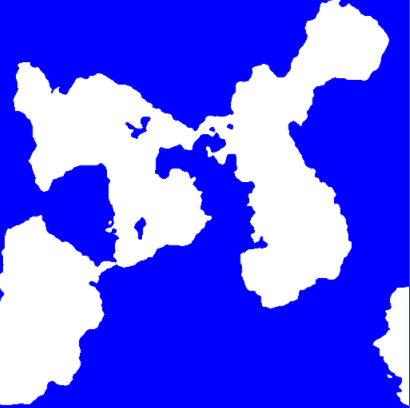Now we are getting somewhere. We can start to see some shapes appear with this simple rule. Let's take this a step further.

Let's add some more adjustable variables to our Generator class. These will define what our height values will assign with.

```	float DeepWater = 0.2f;
float ShallowWater = 0.4f;
float Sand = 0.5f;
float Grass = 0.7f;
float Forest = 0.8f;
float Rock = 0.9f;
float Snow = 1;
```

Let's also add some custom colours to our Texture Generator:

```	private static Color DeepColor = new Color(0, 0, 0.5f, 1);
private static Color ShallowColor = new Color(25/255f, 25/255f, 150/255f, 1);
private static Color SandColor = new Color(240 / 255f, 240 / 255f, 64 / 255f, 1);
private static Color GrassColor = new Color(50 / 255f, 220 / 255f, 20 / 255f, 1);
private static Color ForestColor = new Color(16 / 255f, 160 / 255f, 0, 1);
private static Color RockColor = new Color(0.5f, 0.5f, 0.5f, 1);
private static Color SnowColor = new Color(1, 1, 1, 1);
```

Adding in all these rules, in a similar fashion, and we then get the following results: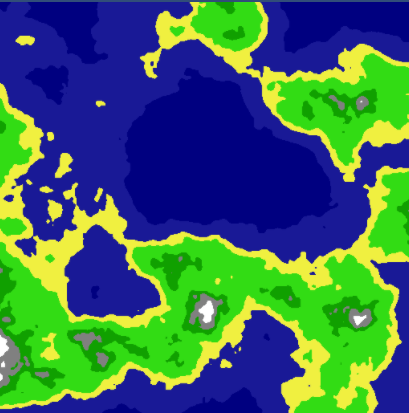Now we have a lovely Height Map, with a nice texture representing it.

You may download the source code on github for part 1 here.

Continue to Part 2 of this series.

## Restricting 2D Camera Zoom and Movement to an Image in Unity C#

The following code will allow you to constrain an orthographic 2D camera, so that it is always contained inside of a background image.

The script will calculate the movement constraints, as well as the max/min zoom values. Regardless of the aspect ratio, the camera will always stay within the image, therefore, never showing any bars on the top or sides of your scene.

The following image, is the demo running, while inside of Scene Mode, to give you an idea of how it works: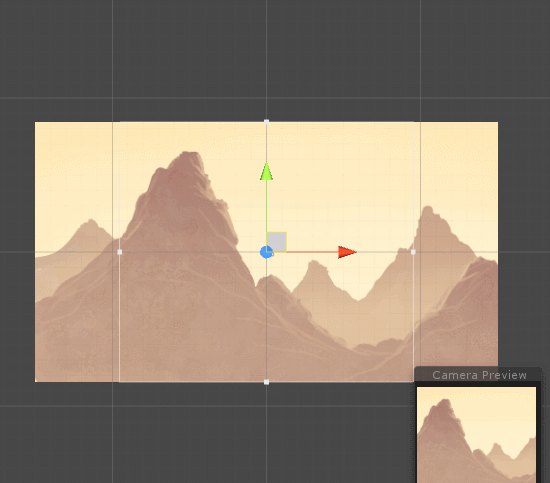The source code is all contained in its own class. Read the comments for extra information:

```using UnityEngine;

public class Camera2D : MonoBehaviour
{
//The background image to use
public Transform Area;

//Sprite details
private Sprite Sprite;
private float PixelUnits;
private Vector2 Size;
private Vector2 Offset;

//Camera bounds
private float Left;
private float Right;
private float Top;
private float Bottom;
private float MaxZoom;
private float MinZoom;

public void Start()
{
Sprite = Area.transform.GetComponent<SpriteRenderer>().sprite;

CalculatePixelUnits();
CalculateSize();
Refresh();
Center();
}

//Calculate the pixel per unit value is for this sprite
private void CalculatePixelUnits()
{
PixelUnits = Sprite.rect.width / Sprite.bounds.size.x;
}

//Calculate the size and offset of the background sprite
private void CalculateSize()
{
Size = new Vector2(Area.transform.localScale.x * Sprite.texture.width / PixelUnits,
Area.transform.localScale.y * Sprite.texture.height / PixelUnits);

Offset = Area.transform.position;
}

//Get zoom constraints, and zoom as large as possible for current view
private void Refresh()
{
//calculate current screen ratio
float w = Screen.width / Size.x;
float h = Screen.height / Size.y;
float ratio = w / h;
float ratio2 = h / w;
if (ratio2 > ratio)
{
MaxZoom = (Size.y / 2);
}
else
{
MaxZoom = (Size.y / 2);
MaxZoom /= ratio;
}

MinZoom = 1;
Camera.main.orthographicSize = MaxZoom;

RefreshBounds();
}

//Position the camera at the center of the background image
private void Center()
{
//set camera to center of background image
Vector2 position = Area.transform.position;
Vector3 camPosition = position;
Vector3 point = Camera.main.WorldToViewportPoint(camPosition);
Vector3 delta = camPosition - Camera.main.ViewportToWorldPoint(new Vector3(0.5f, 0.5f, point.z));
Vector3 destination = transform.position + delta;
transform.position = destination;
}

//Calculate the max distance the camera can travel
private void RefreshBounds()
{
var vertExtent = Camera.main.orthographicSize;
var horzExtent = vertExtent * Screen.width / Screen.height;

Left = horzExtent - Size.x / 2.0f + Offset.x;
Right = Size.x / 2.0f - horzExtent + Offset.x;
Bottom = vertExtent - Size.y / 2.0f + Offset.y;
Top = Size.y / 2.0f - vertExtent + Offset.y;
}

public void Update()
{
//You would typically hook into Refresh on a screen rotation or aspect ratio change
//In demo, we call it non stop to demonstrate the camera system
Refresh();
}

public void LateUpdate()
{
//Clamp camera inside of our bounds
Vector3 v3 = transform.position;
v3.x = Mathf.Clamp(v3.x, Left, Right);
v3.y = Mathf.Clamp(v3.y, Bottom, Top);
transform.position = v3;
}

public void Zoom(float value)
{
Camera.main.orthographicSize += value;
Camera.main.orthographicSize = Mathf.Clamp(Camera.main.orthographicSize, MinZoom, MaxZoom);
}
}
```

The above script can be attached to a Orthographic Camera. The last thing you need to do is attach a GameObject that contains a SpriteRenderer to the newly added script: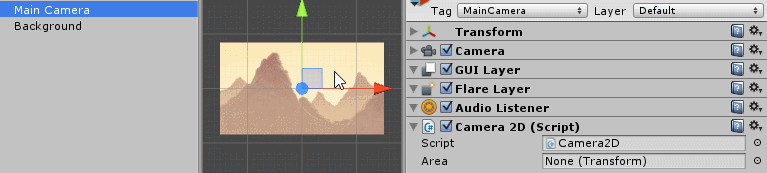## Nodal Pathfinding in Unity 2D with A* in Non Grid Based Games

In a typical 2D grid based game, you restrict your level to fit into predefined squares. This makes it pretty easy to process this data with an A* pathfinding algorithm. But what happens if you are not creating tile-based levels?

Consider a level that resembles the following: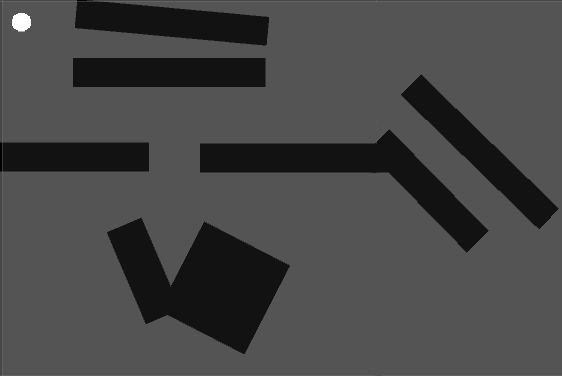The black squares are walls. The white circle is our movable character. Our goal is to provide a mechanism that will allow us to calculate A* paths in a Unity level such as this.

The first step, is to define an area that we will use to generate and calculate the nodal map. In this demo we do this by creating a GameObject (Grid gameobject), and setting its position and scale. This will be the area we will use to generate our Nodes.

These Nodes will serve as way points to help your character maneuver the map. Once these way points are generated, it resembles like this: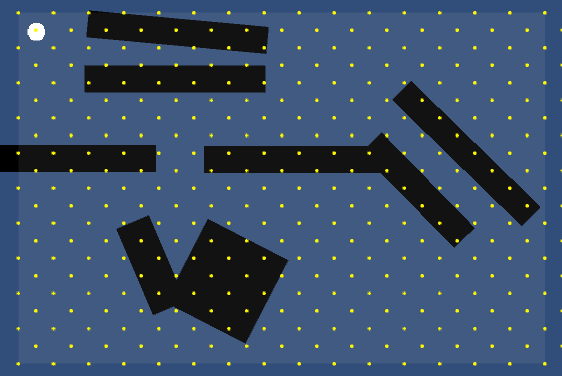I opted to generate the nodes in a diamond shaped pattern, as it provides a much better nodal mesh than a square pattern.

The distance between each node also matter. You need to match up your character’s size with the distance between nodes, otherwise you will not know if your character will be able to fit in between some holes. As long as your character is smaller than a half-unit, it will be able to fit. You could always generate multiple maps, if you have characters that vary greatly in size.

Here is how I setup my unit size for the demo. This circle should be able to pass between any two nodes: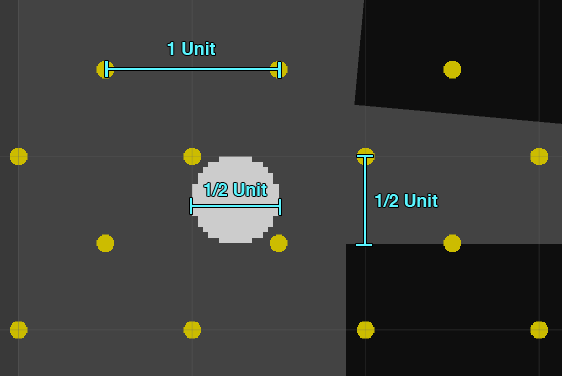The next step is to generate the Connections between each node. For each Node, we raycast in all 8 directions, to determine if the path if valid or not. If the raycast hits a wall, or if the raycast is traveling outside of the defined area, then we mark the connection as bad (red). If the check passes, we mark the connection as valid (green). Each Node will then have 8 connections.

This gives us our starting connection map that resembles this: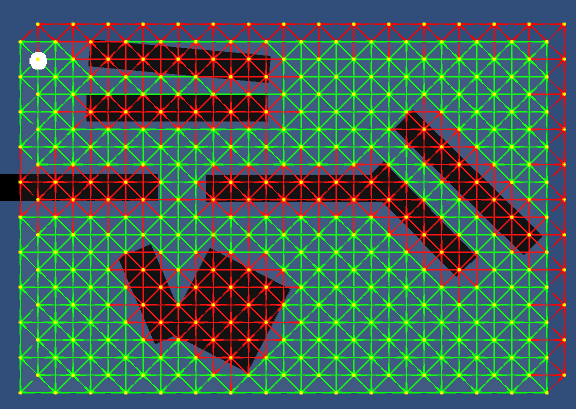Removing the red lines from our output, we can then look at the valid paths that were generated from this simple base check: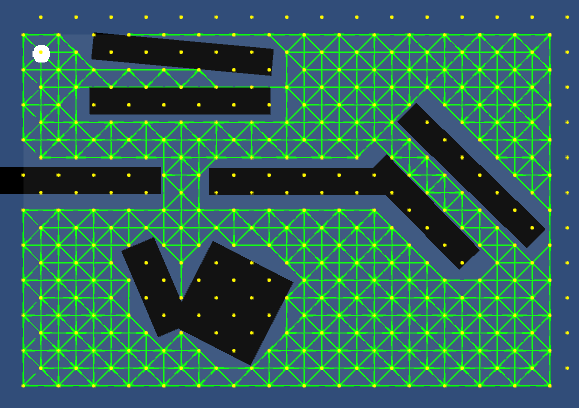You can immediately notice that some of the paths are bad, as the circle cannot navigate between the two top-most walls for example. Also, there are some paths that lead to dead-ends, which we don’t want. We need to cull these bad paths, in order for our pathfinder to work properly.

We first start culling some Nodes, based on its connections. If a Node does not have at least 3 valid connection points, we remove them. In the following image, we mark these Nodes by coloring them red: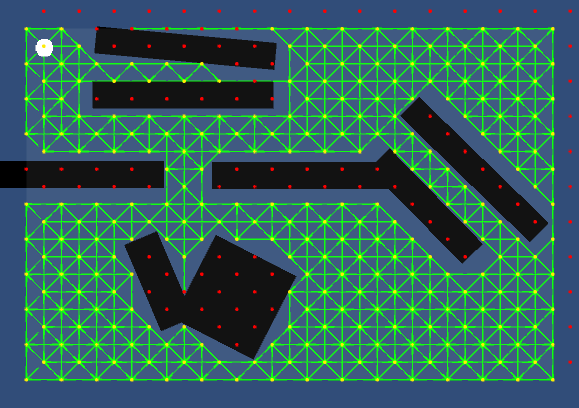Now that we have all of the bad Nodes, we remove any of the connections traveling to and from them. This gives us a much nicer Nodal map than what we had previously: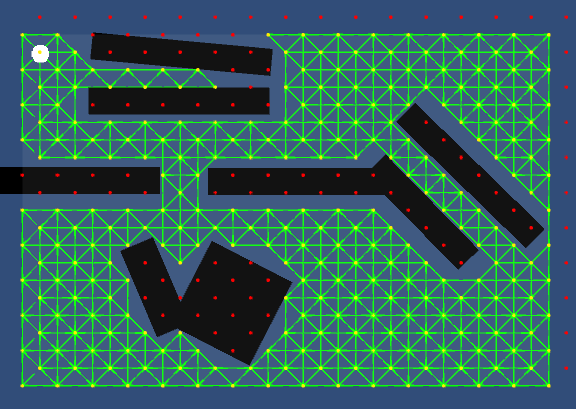Then it is just a matter of plugging in each connection as a neighbor in your A* pathfinding algorithm. Your A* neighbors are all defined by your Nodes and their Connections.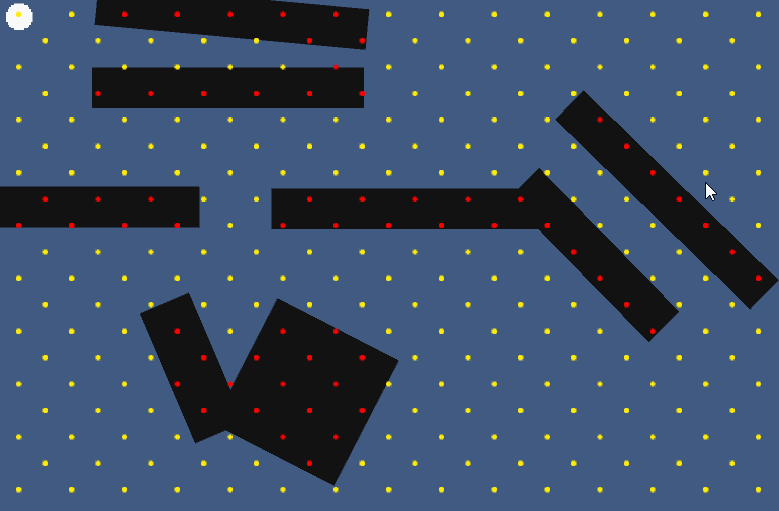Feel free to try the Demo.

### Update:

A friend of mine has expanded on this nodal pathfinding model, and has pusblished his code over on his Github

Example gif

## Procedurally Generated Trees with Space Colonization Algorithm in XNA C#

I discovered a very interesting algorithm called “Space Colonization Algorithm”, which is described in a paper found here:

http://algorithmicbotany.org/papers/colonization.egwnp2007.html

With this algorithm, it is possible to create some pretty lifelike trees. With L-Systems, you can get some interesting looking trees, which typically start from the root, and generates branches based on a set of rules. The Space Colonization algorithm takes a different approach to the problem. Instead you first create the leaves, which serve as attraction points for the branches. Over each iteration, the branches grow closer and closer towards the leaves. In this manner, you end up having tree branches that appear to have grown towards some light source.

I decided to write the algorithm in C#. The following is an example of a tree that was generated using this algorithm: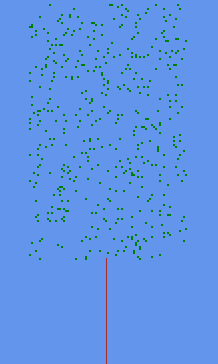The algorithm works as follows:

1. Define an area for the crown of the tree. In our examples, we are using Rectangles, but any shape can be used. In fact, very interesting trees can be created by using various shapes.
2. Populate the defined area with attraction points (I call these leaves).
3. Create the trunk of the tree, by adding Branches below the defined area. Keep growing branches upwards until the MaxDistance between a Leaf and a Branch is reached. This MaxDistance is a parameter that defines how far a Leaf can be to attract a Branch. A Branch is not affected by Leaves that are further away than MaxDistance.
4. At this point, your tree would look something like this, and is now ready to grow: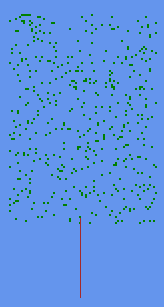5. Process the Leaves, by comparing it to all the Branches. Calculate the direction and distance from the Leaf to the Branch. If the distance is smaller than MinDistance, we remove the Leaf for it has been reached. If the distance is greater than MaxDistance, we ignore it, since it is too far. Otherwise, we check if the Branch is the closest Branch to this Leaf. Each Leaf can only affect 1 Branch at a time.
6. Once the closest Branch is determined, we increment the GrowCount of that Branch, and add the direction of the Leaf to the GrowDirection of the Branch. If multiple Leaves attract a branch, then the GrowDirection will be an average of all of the Leaf directions.
7. Now loop through the Branches, and process any Branch with a GrowCount > 0. Divide the GrowDirection by the GrowCount, to get the average direction, then create a new branch with this GrowDirection, linking it to the Branch being processed as its parent. Then reset the GrowCount and GrowDirection of the parent Branch.
8. Repeat from step 4 until there are no Leaves left, or no more Branches are growing.

To help visualize what is happening during each iteration, have a look at the following image: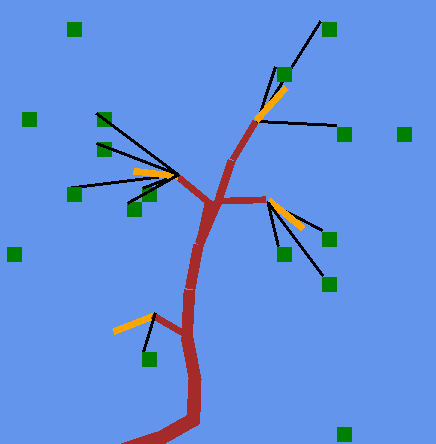The green squares are Leaves. The brown Branches are Branches that were grown in previous iterations. The black lines indicate each Leaf’s attraction to its nearest Branches (that are in range). The orange lines indicate the Branches that will grow in this iteration based on those attraction points.

The orange Branches will grow with respect to the original parent Branch GrowDirection, adding the attraction points average direction to this value.

Onto the code. See the bottom of the page for a download of a sample project for Visual Studio.

Leaf class:
```public class Leaf
{
public Vector2 Position { get; set; }
public Branch ClosestBranch { get; set; }

public Leaf(Vector2 position)
{
Position = position;
}
}
```
Branch class:
```public class Branch
{
public Branch Parent { get; private set; }
public Vector2 GrowDirection { get; set; }
public Vector2 OriginalGrowDirection { get; set; }
public int GrowCount { get; set; }
public Vector2 Position { get; private set; }

public Branch(Branch parent, Vector2 position, Vector2 growDirection)
{
Parent = parent;
Position = position;
GrowDirection = growDirection;
OriginalGrowDirection = growDirection;
}

public void Reset()
{
GrowCount = 0;
GrowDirection = OriginalGrowDirection;
}
}
```
Tree class:
```public class Tree
{
bool DoneGrowing = false;

Vector2 Position = Vector2.Zero;

int LeafCount = 400;
int TreeWidth = 80;
int TreeHeight = 150;
int TrunkHeight = 40;
int MinDistance = 2;
int MaxDistance = 15;
int BranchLength = 2;

Branch Root;
List<Leaf> Leaves;
Dictionary<Vector2, Branch> Branches;

Rectangle Crown;

public Tree(Vector2 position)
{
Position = position;
GenerateCrown();
GenerateTrunk();
}

private void GenerateCrown()
{
Crown = new Rectangle((int)Position.X - TreeWidth / 2, (int)Position.Y - TreeHeight - TrunkHeight, TreeWidth, TreeHeight);
Leaves = new List<Leaf>();

//randomly place leaves within our rectangle
for (int i = 0; i < LeafCount; i++)
{
Vector2 location = new Vector2(Random.Next(Crown.Left, Crown.Right + 1), Random.Next(Crown.Top, Crown.Bottom + 1));
Leaf leaf = new Leaf(location);
}
}

private void GenerateTrunk()
{
Branches = new Dictionary<Vector2, Branch>();

Root = new Branch(null, Position, new Vector2(0, -1));

Branch current = new Branch(Root, new Vector2(Position.X, Position.Y - BranchLength), new Vector2(0, -1));

//Keep growing trunk upwards until we reach a leaf
while ((Root.Position - current.Position).Length() < TrunkHeight)
{
Branch trunk = new Branch(current, new Vector2(current.Position.X, current.Position.Y - BranchLength), new Vector2(0, -1));
current = trunk;
}
}

public void Grow()
{
if (DoneGrowing) return;

//If no leaves left, we are done
if (Leaves.Count == 0) {
DoneGrowing = true;
return;
}

//process the leaves
for (int i = 0; i < Leaves.Count; i++)
{
bool leafRemoved = false;

Leaves[i].ClosestBranch = null;
Vector2 direction = Vector2.Zero;

//Find the nearest branch for this leaf
foreach (Branch b in Branches.Values)
{
direction = Leaves[i].Position - b.Position;                       //direction to branch from leaf
float distance = (float)Math.Round(direction.Length());            //distance to branch from leaf
direction.Normalize();

if (distance <= MinDistance)            //Min leaf distance reached, we remove it
{
Leaves.Remove(Leaves[i]);
i--;
leafRemoved = true;
break;
}
else if (distance <= MaxDistance)       //branch in range, determine if it is the nearest
{
if (Leaves[i].ClosestBranch == null)
Leaves[i].ClosestBranch = b;
else if ((Leaves[i].Position - Leaves[i].ClosestBranch.Position).Length() > distance)
Leaves[i].ClosestBranch = b;
}
}

//if the leaf was removed, skip
if (!leafRemoved)
{
//Set the grow parameters on all the closest branches that are in range
if (Leaves[i].ClosestBranch != null)
{
Vector2 dir = Leaves[i].Position - Leaves[i].ClosestBranch.Position;
dir.Normalize();
Leaves[i].ClosestBranch.GrowDirection += dir;       //add to grow direction of branch
Leaves[i].ClosestBranch.GrowCount++;
}
}
}

//Generate the new branches
HashSet<Branch> newBranches = new HashSet<Branch>();
foreach (Branch b in Branches.Values)
{
if (b.GrowCount > 0)    //if at least one leaf is affecting the branch
{
Vector2 avgDirection = b.GrowDirection / b.GrowCount;
avgDirection.Normalize();

Branch newBranch = new Branch(b, b.Position + avgDirection * BranchLength, avgDirection);

b.Reset();
}
}

//Add the new branches to the tree
foreach (Branch b in newBranches)
{
//Check if branch already exists.  These cases seem to happen when leaf is in specific areas
Branch existing;
if (!Branches.TryGetValue(b.Position, out existing))
{
}
}

//if no branches were added - we are done
//this handles issues where leaves equal out each other, making branches grow without ever reaching the leaf
DoneGrowing = true;
}
}
```
With further improvements and refinements, you can make the tree form any shape you want: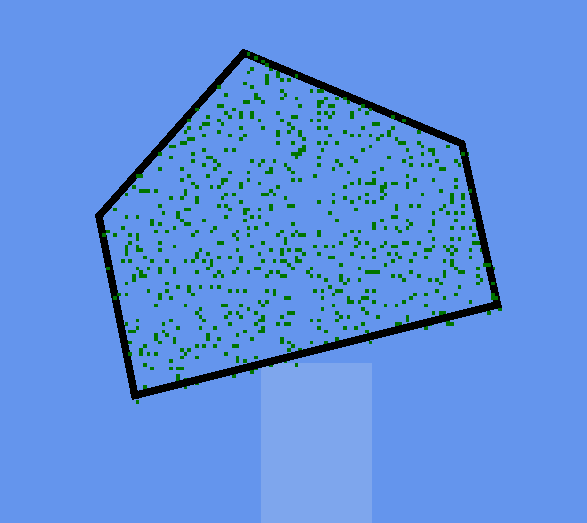Rendering some leaves: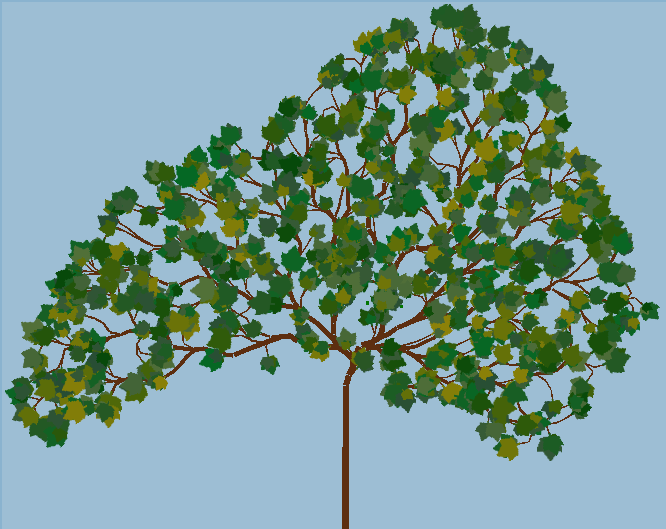Here is a gif to demonstrate what is possible with this method:
Tree Growing Gif

Cycling through the 4 seasons:
Season Cycle Gif

The demo project can be downloaded here. I have omitted the polygon code for the sake of simplicity, the trees are set to be Rectangles only.

http://www.jgallant.com/files/TreeGenerator.zip

Feel free to contact me if you have any questions or comments.

References used:
http://algorithmicbotany.org/papers/colonization.egwnp2007.html
http://procworld.blogspot.ca/2011/02/space-colonization.html
http://www.sea-of-memes.com/LetsCode26/LetsCode26.html

## Auto calculating bounding box from texture in Monogame / XNA

My current project involves thousands of frames of animation. If I were to determine the bounding boxes of all these frames by hand, it would be very tedious. Instead, I decided to auto calculate all the bounding boxes. To better illustrate this, take a look at the following animation: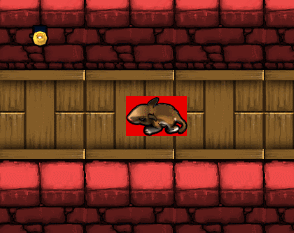Notice how the red portion (bounding box) automatically re-sizes based on the current frame of the animation. Having these dynamic bounding boxes helps greatly when dealing with collision detection and collision response.

The idea behind this is quite simple. When loading your images into your game, you calculate the smallest rectangle possible, based on the pixel data. If a pixel is transparent, we do not include it in our rectangle, otherwise, we do. Take a look at the following code:
```public static class CalculateBounds
{
//Get smallest rectangle from Texture, cased on color
public static Rectangle GetSmallestRectangleFromTexture(Texture2D Texture)
{
//Create our index of sprite frames
Color[,] Colors = TextureTo2DArray(Texture);

//determine the min/max bounds
int x1 = 9999999, y1 = 9999999;
int x2 = -999999, y2 = -999999;

for (int a = 0; a < Texture.Width; a++)
{
for (int b = 0; b < Texture.Height; b++)
{
//If we find a non transparent pixel, update bounds if required
if (Colors[a, b].A != 0)
{
if (x1 > a) x1 = a;
if (x2 < a) x2 = a;

if (y1 > b) y1 = b;
if (y2 < b) y2 = b;
}
}
}

//We now have our smallest possible rectangle for this texture
return new Rectangle(x1, y1, x2 - x1 + 1, y2 - y1 + 1);
}

//convert texture to 2d array
private static Color[,] TextureTo2DArray(Texture2D texture)
{
//Texture.GetData returns a 1D array
Color[] colors1D = new Color[texture.Width * texture.Height];
texture.GetData(colors1D);

//convert the 1D array to 2D for easier processing
Color[,] colors2D = new Color[texture.Width, texture.Height];
for (int x = 0; x < texture.Width; x++)
for (int y = 0; y < texture.Height; y++)
colors2D[x, y] = colors1D[x + y * texture.Width];

return colors2D;
}
}
```

You can further optimize by running the calculations once, and saving the bounds data to file. Then load the pre-calculated data when you run your game.

Contact me with any questions or comments.

## How to Create a Parallax Effect in Monogame

This post will explain how to create a parallax effect in Monogame. First, take a look at the demo video to see what we will be doing:

This effect can be accomplished quite easily in Monogame. We create a Background class, so that we can layer multiple backgrounds together. This class will keep track of individual background images, speed, and zoom level.

Have a look at the documented code below:
```using Microsoft.Xna.Framework.Graphics;
using Microsoft.Xna.Framework;

namespace MonogameParallax
{
public class Background
{
private Texture2D Texture;      //The image to use
private Vector2 Offset;         //Offset to start drawing our image
public Vector2 Speed;           //Speed of movement of our parallax effect
public float Zoom;              //Zoom level of our image

private Viewport Viewport;      //Our game viewport

//Calculate Rectangle dimensions, based on offset/viewport/zoom values
private Rectangle Rectangle
{
get { return new Rectangle((int)(Offset.X), (int)(Offset.Y), (int)(Viewport.Width / Zoom), (int)(Viewport.Height / Zoom)); }
}

public Background(Texture2D texture, Vector2 speed, float zoom)
{
Texture = texture;
Offset = Vector2.Zero;
Speed = speed;
Zoom = zoom;
}

public void Update(GameTime gametime, Vector2 direction, Viewport viewport)
{
float elapsed = (float)gametime.ElapsedGameTime.TotalSeconds;

//Store the viewport
Viewport = viewport;

//Calculate the distance to move our image, based on speed
Vector2 distance = direction * Speed * elapsed;

//Update our offset
Offset += distance;
}

public void Draw(SpriteBatch spriteBatch)
{
spriteBatch.Draw(Texture, new Vector2(Viewport.X, Viewport.Y), Rectangle, Color.White, 0, Vector2.Zero, Zoom, SpriteEffects.None, 1);
}
}
}```
In order for these Backgrounds to fill our entire viewport area, they need to be drawn using a LinearWrap SamplerState. The SampleState is specified during our SpriteBatch initialization.

Since the background textures are drawn to wrap, we create the illusion of moving the image by setting an Offset point to begin drawing our texture. This offset is manipulated by a Direction vector during the Update call.

I have provided a main Game class to demonstrate an implementation of this technique:
```using Microsoft.Xna.Framework;
using Microsoft.Xna.Framework.Graphics;
using System.Collections.Generic;
using Microsoft.Xna.Framework.Input;

namespace MonogameParallax
{
public class MonogameParallax : Game
{
GraphicsDeviceManager graphics;
SpriteBatch spriteBatch;
List<Background> Backgrounds;

public MonogameParallax() : base()
{
graphics = new GraphicsDeviceManager(this);
Content.RootDirectory = "Content";
}

protected override void Initialize()
{
base.Initialize();
}

{
spriteBatch = new SpriteBatch(GraphicsDevice);

Backgrounds = new List<Background>();
}

{
}

protected override void Update(GameTime gameTime)
{
KeyboardState kbState = Keyboard.GetState();

if (GamePad.GetState(PlayerIndex.One).Buttons.Back == ButtonState.Pressed || Keyboard.GetState().IsKeyDown(Keys.Escape))
Exit();

//Get directional vector based on keyboard input
Vector2 direction = Vector2.Zero;
if (kbState.IsKeyDown(Keys.Up))
direction = new Vector2(0, -1);
else if (kbState.IsKeyDown(Keys.Down))
direction = new Vector2(0, 1);
if (kbState.IsKeyDown(Keys.Left))
direction += new Vector2(-1, 0);
else if (kbState.IsKeyDown(Keys.Right))
direction += new Vector2(1, 0);

//Update backgrounds
foreach (Background bg in Backgrounds)
bg.Update(gameTime, direction, GraphicsDevice.Viewport);

base.Update(gameTime);
}

protected override void Draw(GameTime gameTime)
{
GraphicsDevice.Clear(Color.CornflowerBlue);

//Draw our parallax backgrounds, using a Linear Wrap
spriteBatch.Begin(SpriteSortMode.Deferred, null, SamplerState.LinearWrap, null, null);
foreach (Background bg in Backgrounds)
bg.Draw(spriteBatch);
spriteBatch.End();

base.Draw(gameTime);
}
}
}```